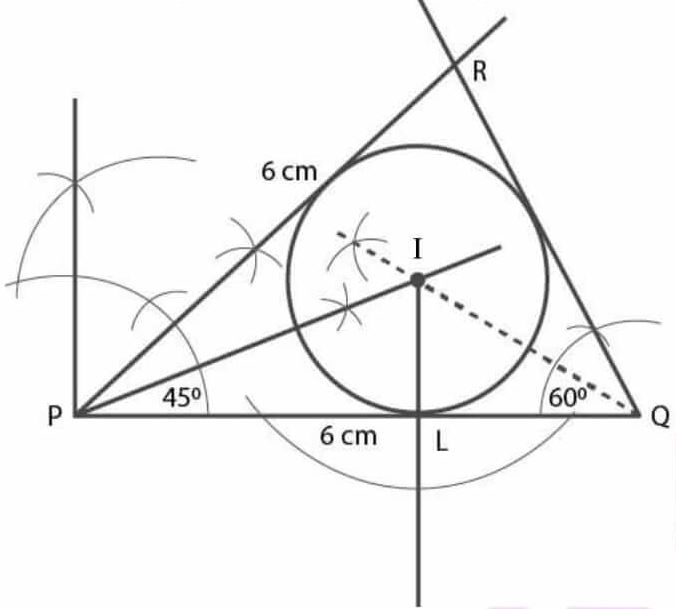# Selina Solutions Concise Maths Class 7 Chapter 15: Triangles

Selina Solutions Concise Maths Class 7 Chapter 15 Triangles provides students with a clear idea about the basic concepts covered in this chapter. The solutions are prepared by faculty after conducting research on each topic, keeping in mind the understanding capacity of students. Here, the students can download Selina Solutions Concise Maths Class 7 Chapter 15 Triangles free PDF, from the links which are provided here.

Chapter 15 helps students understand the types of triangles based on the angles and length of sides. The solutions improve problem solving and analytical thinking skills among students, which are important from the exam point of view.

## Selina Solutions Concise Maths Class 7 Chapter 15: Triangles Download PDF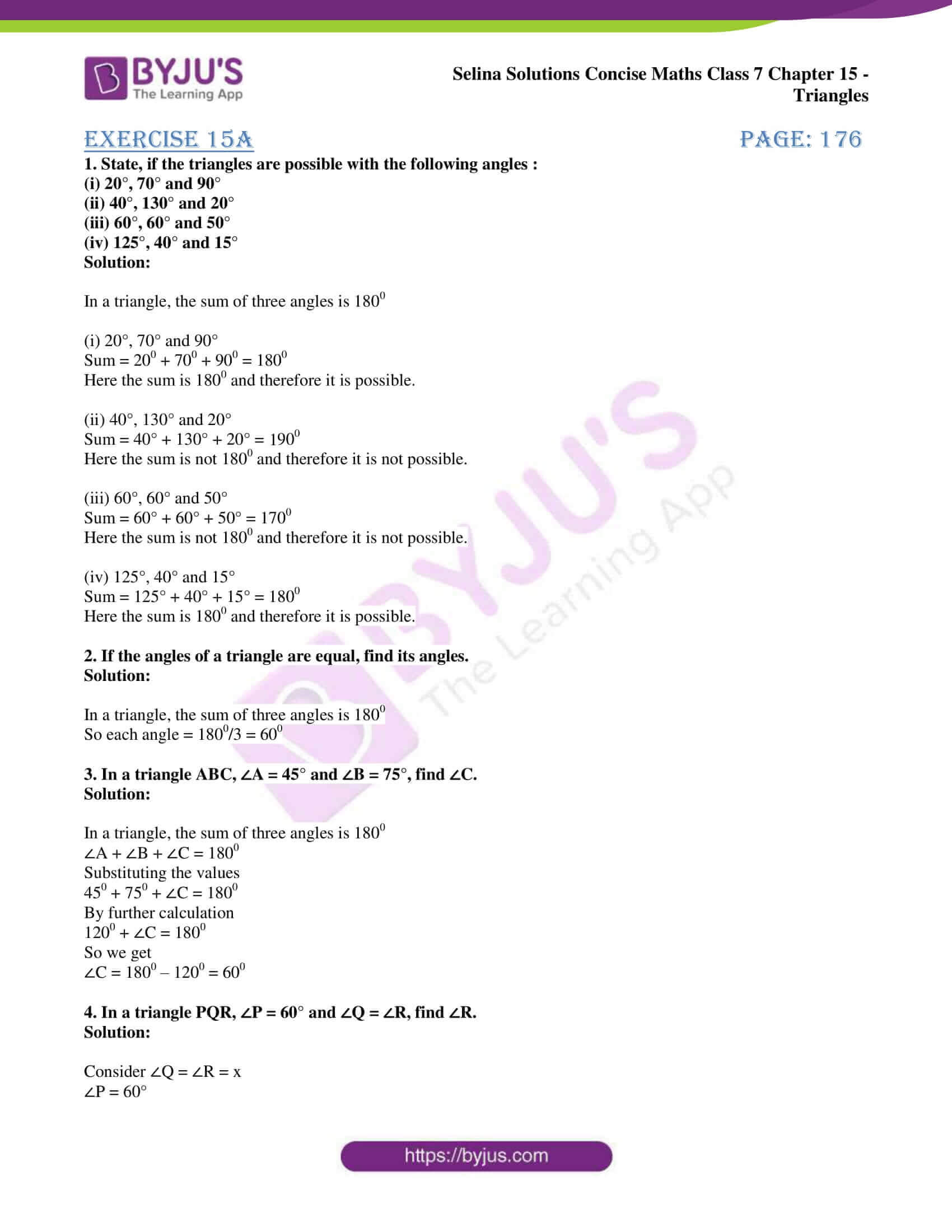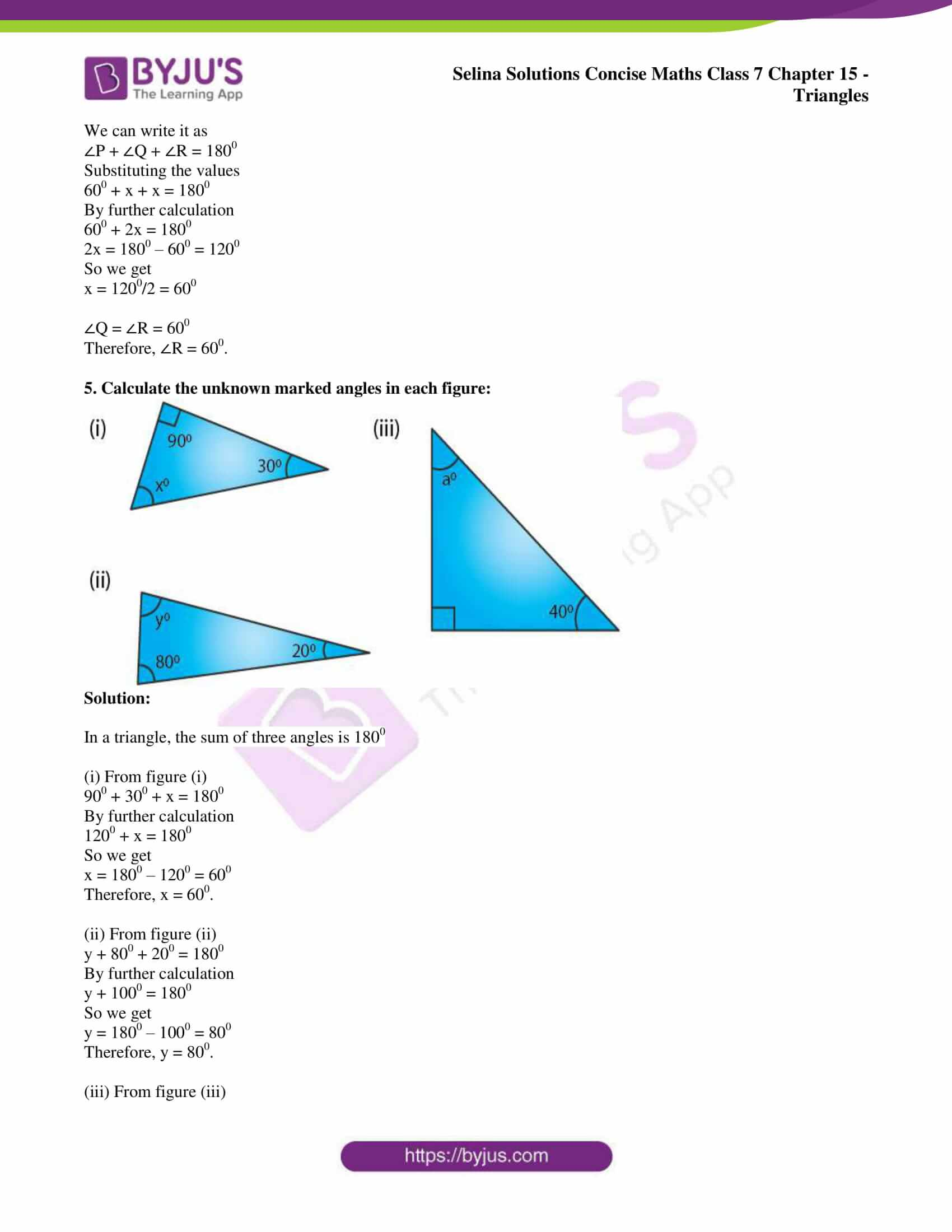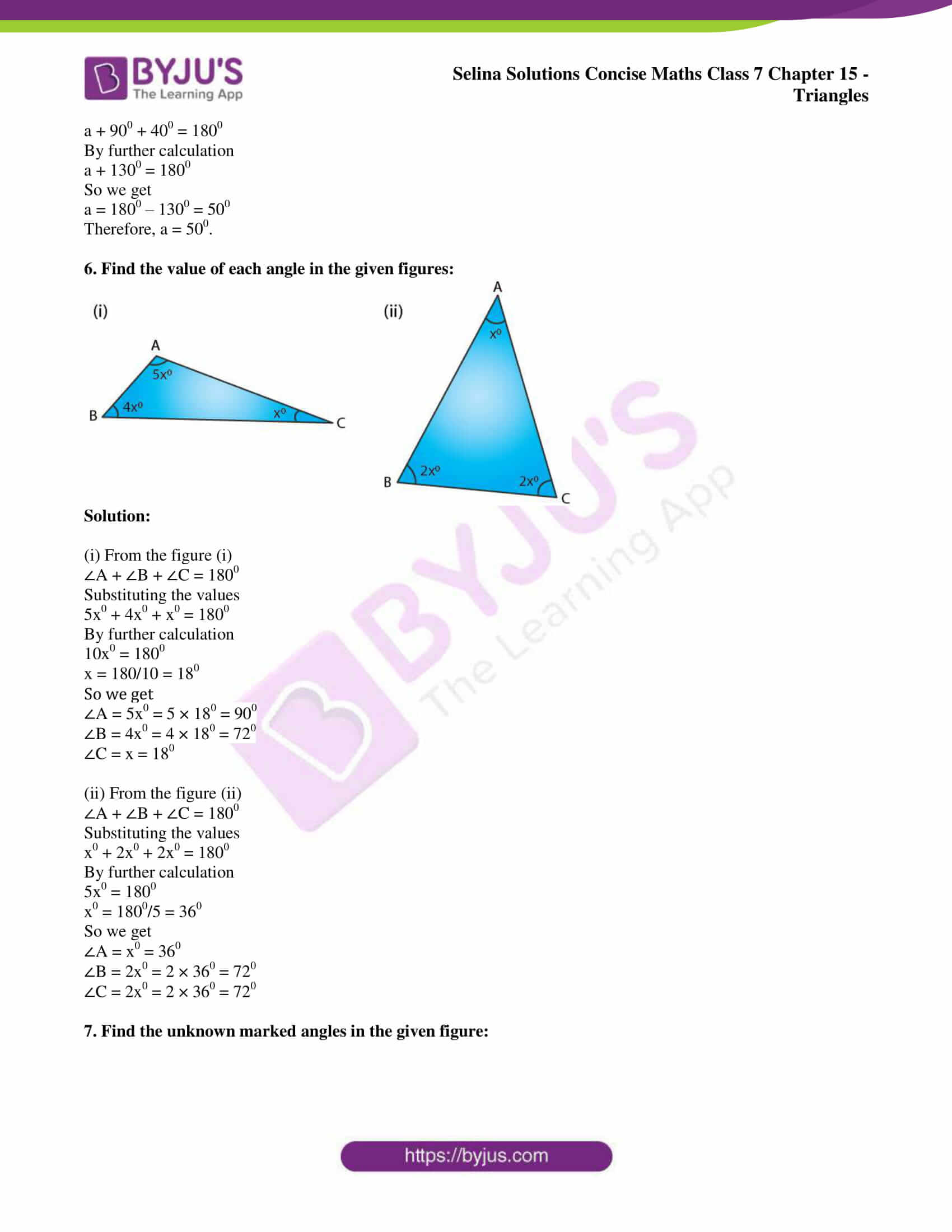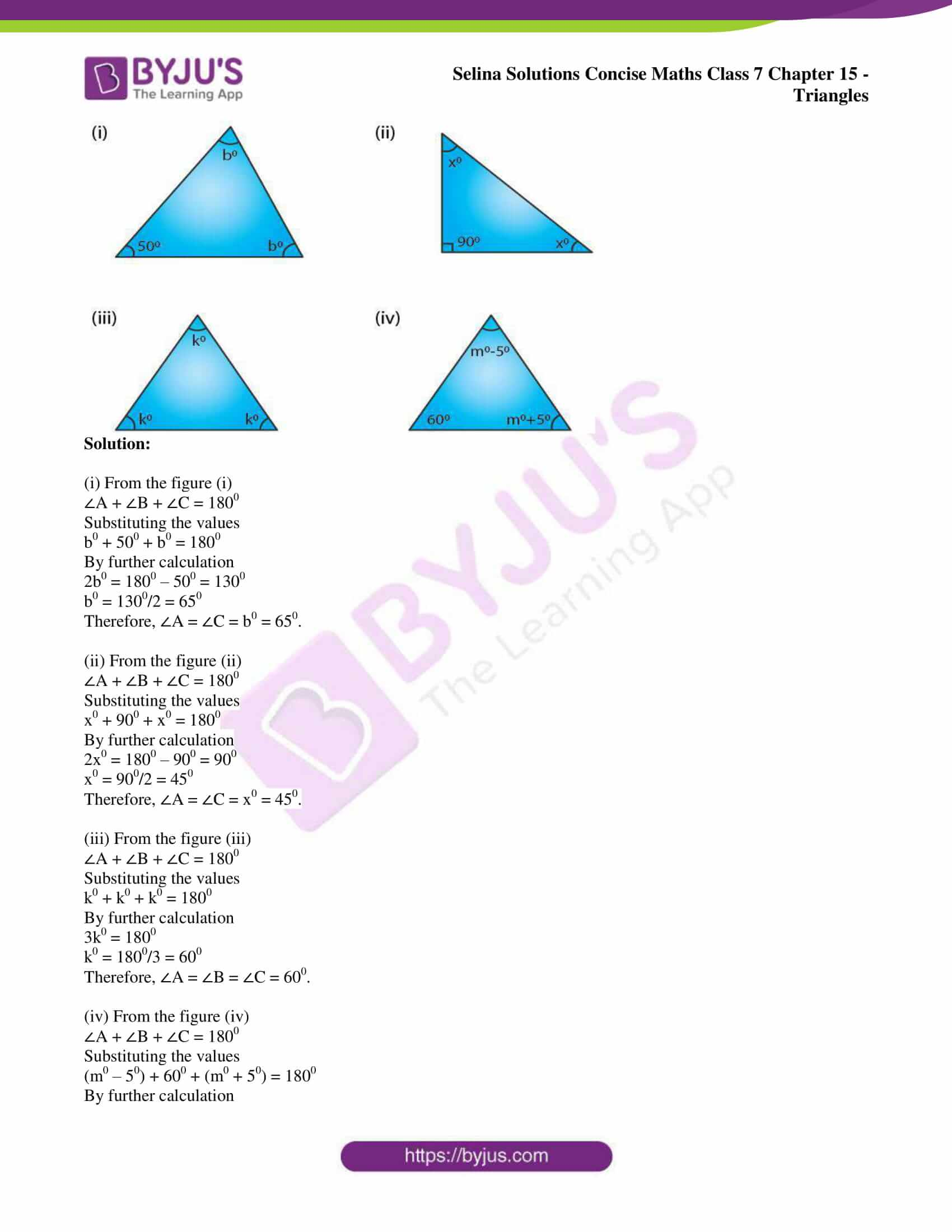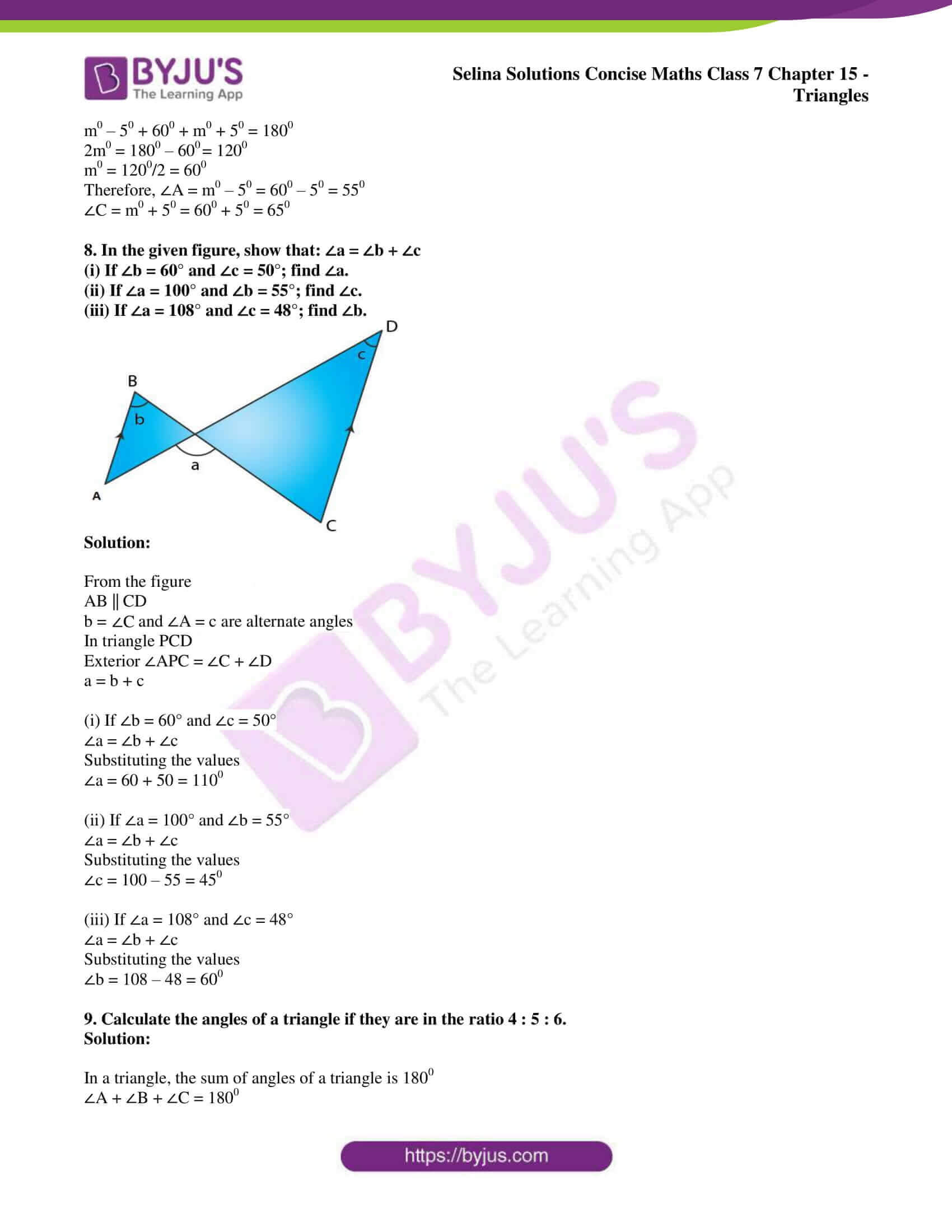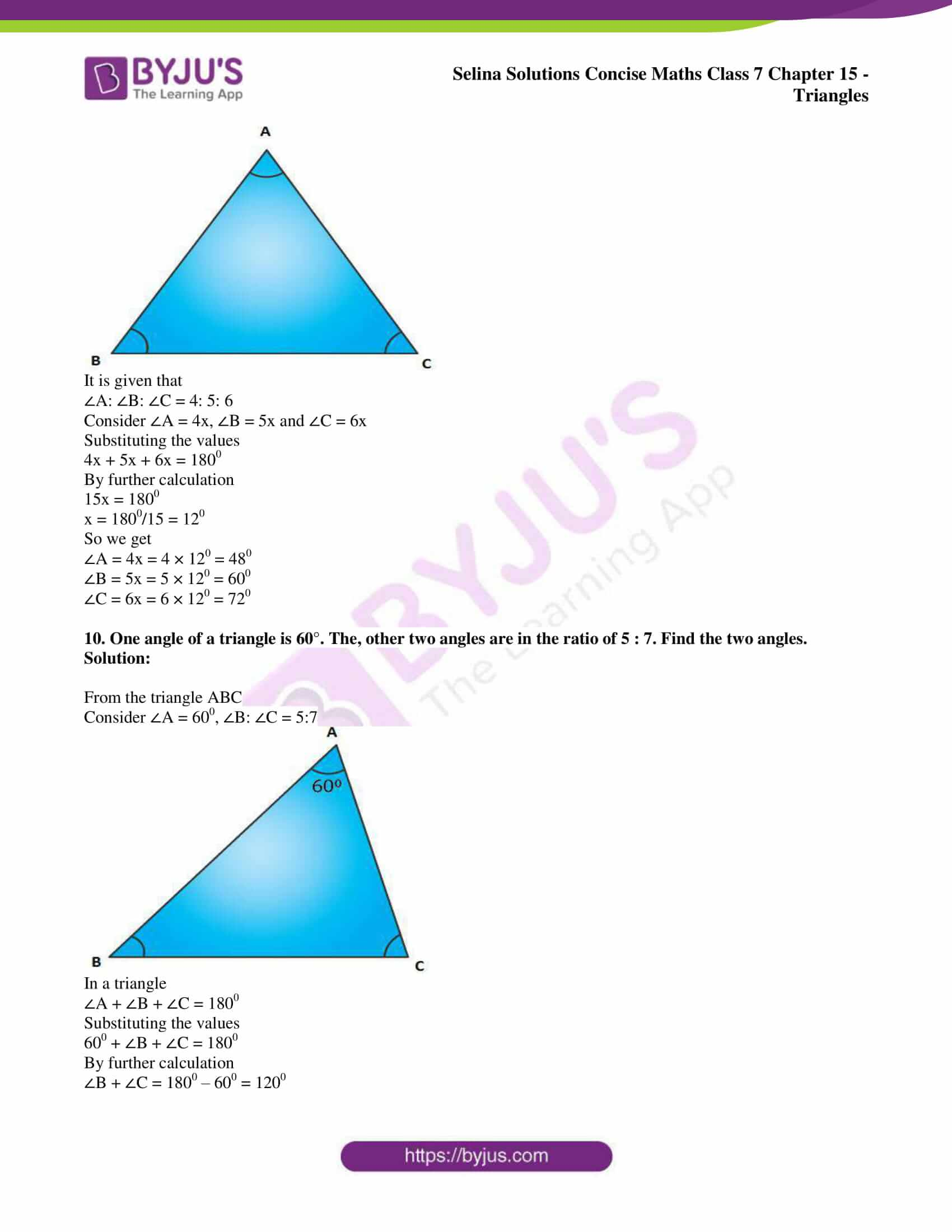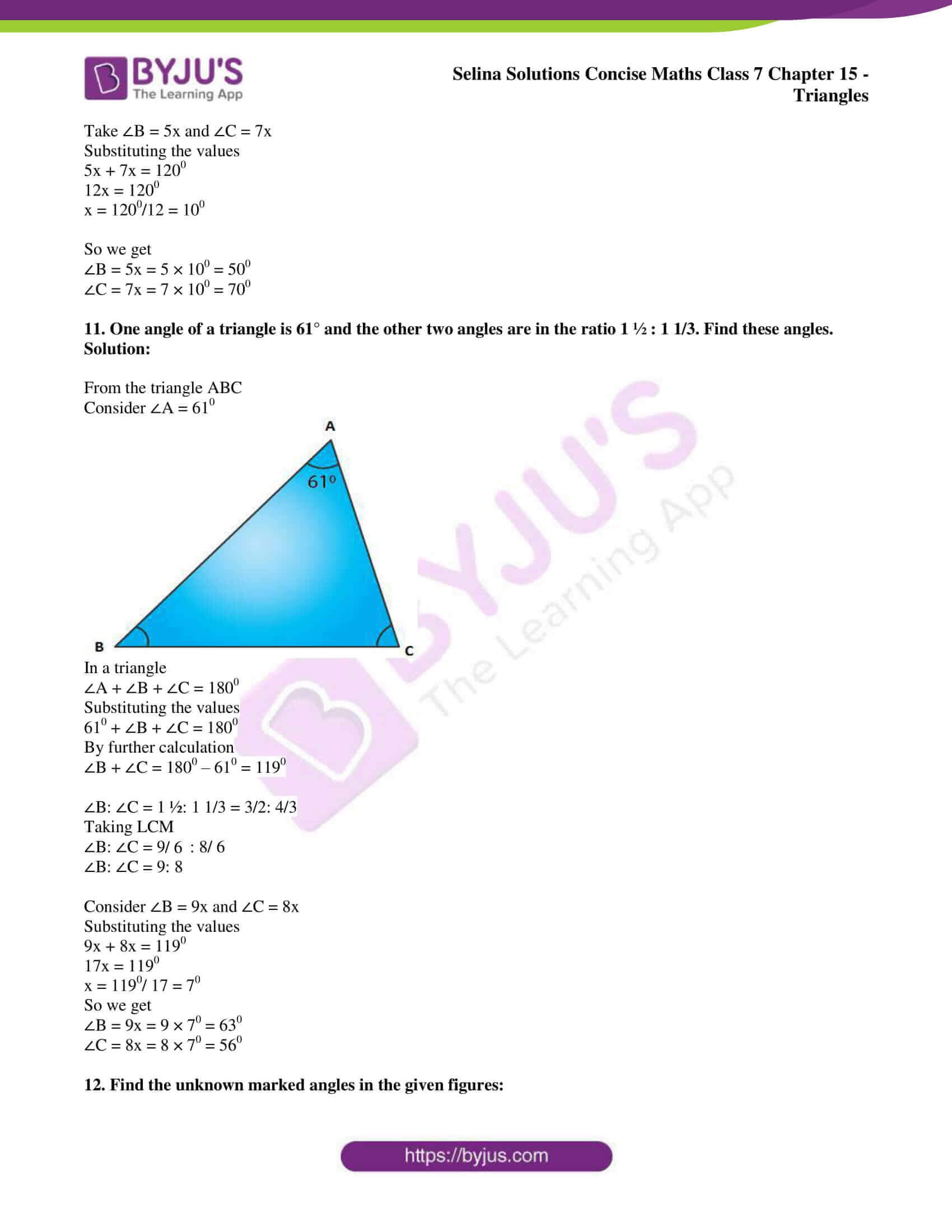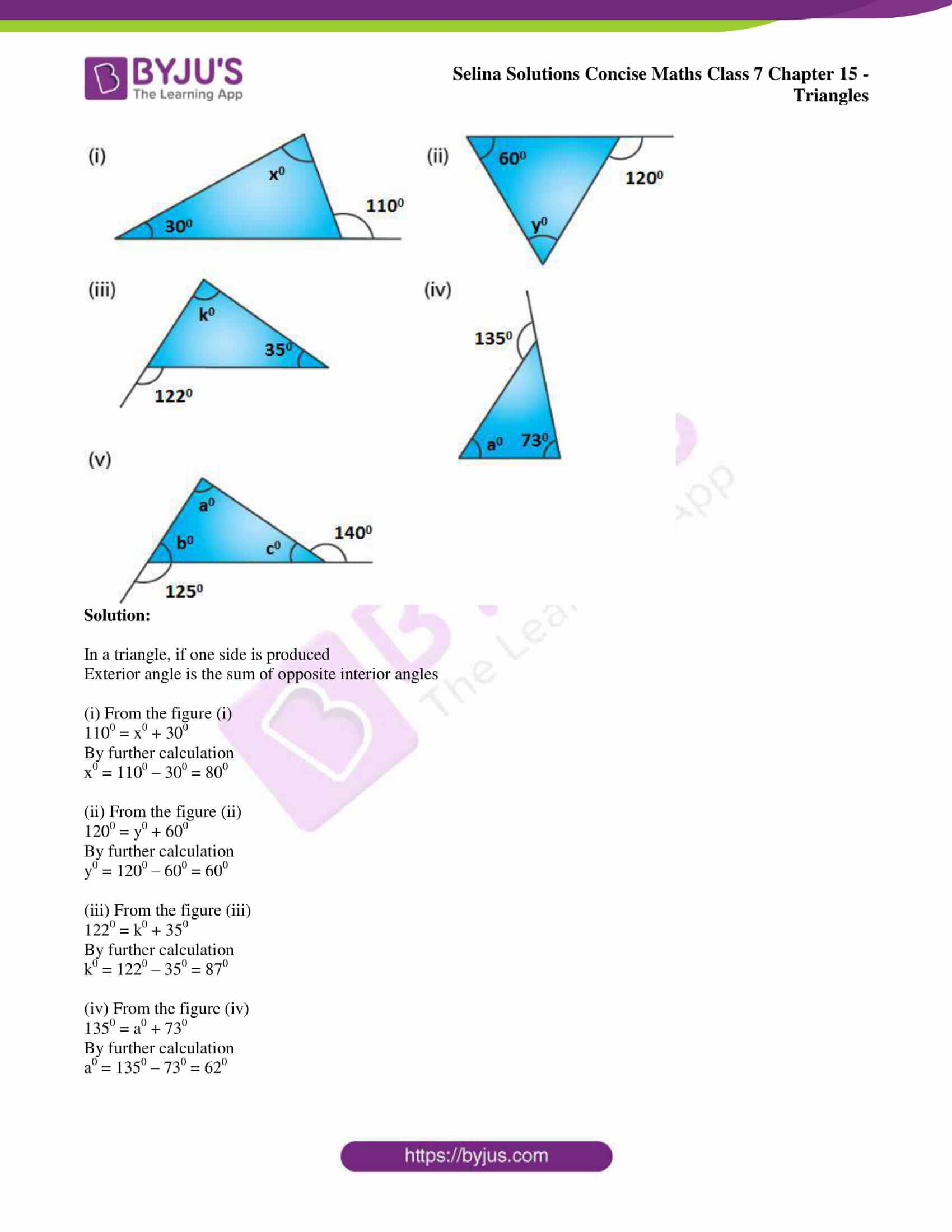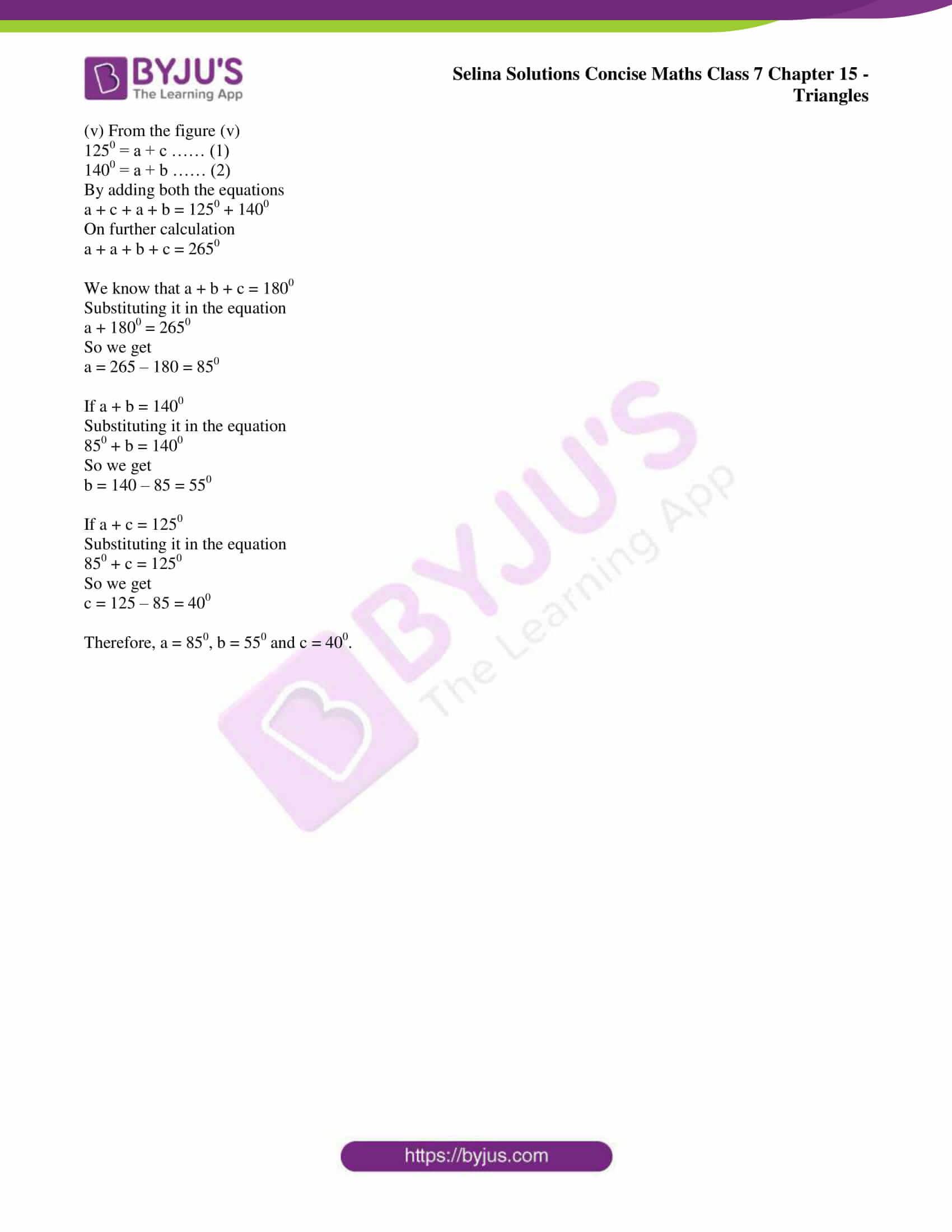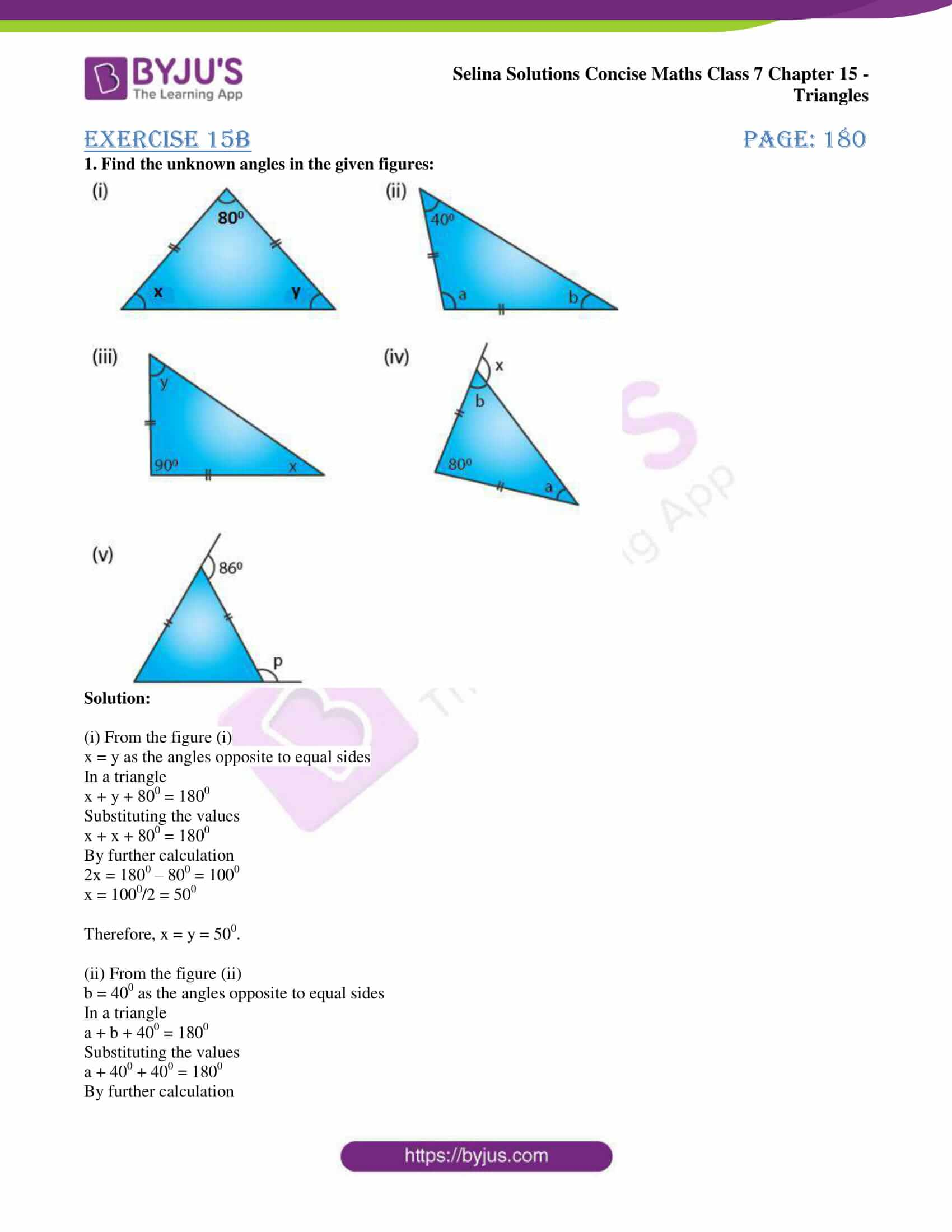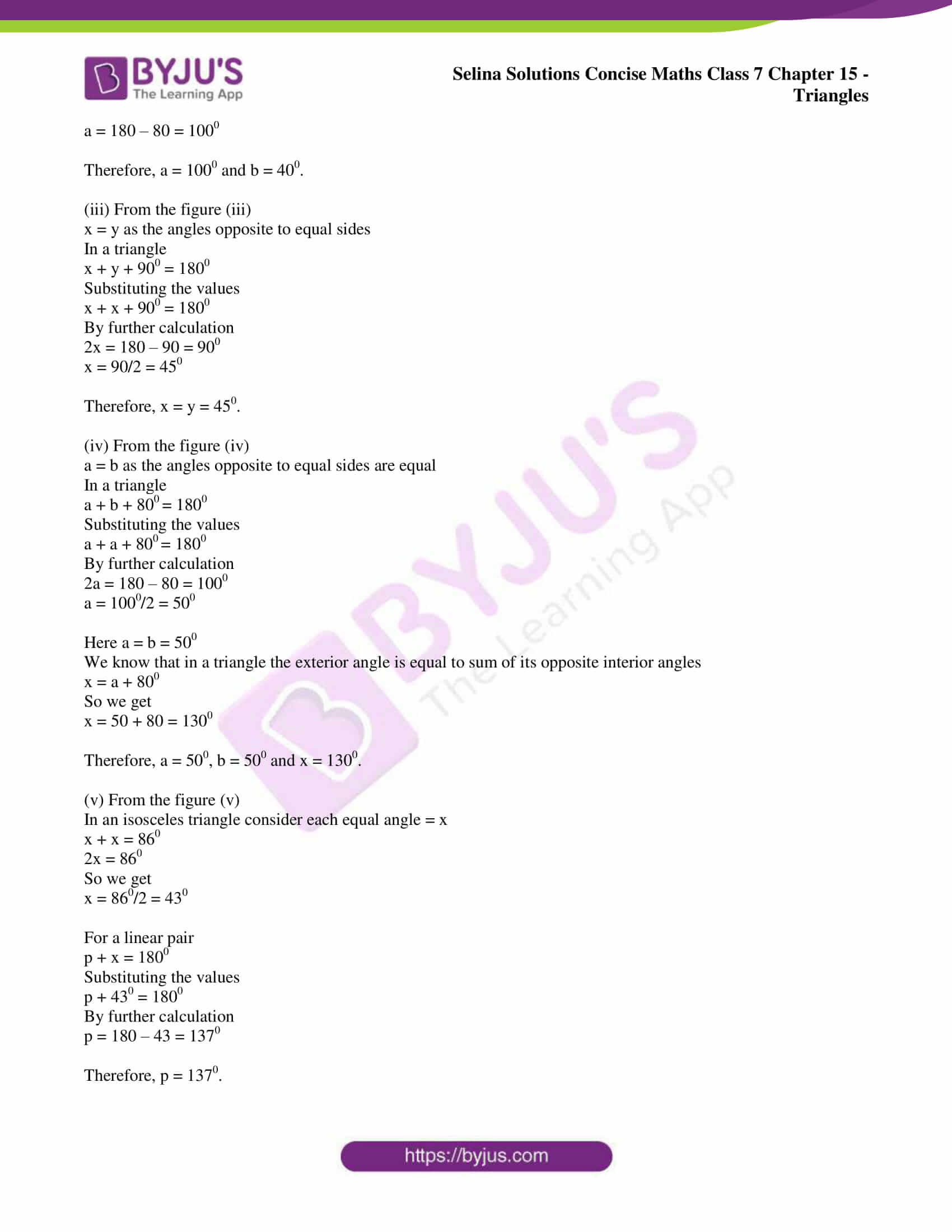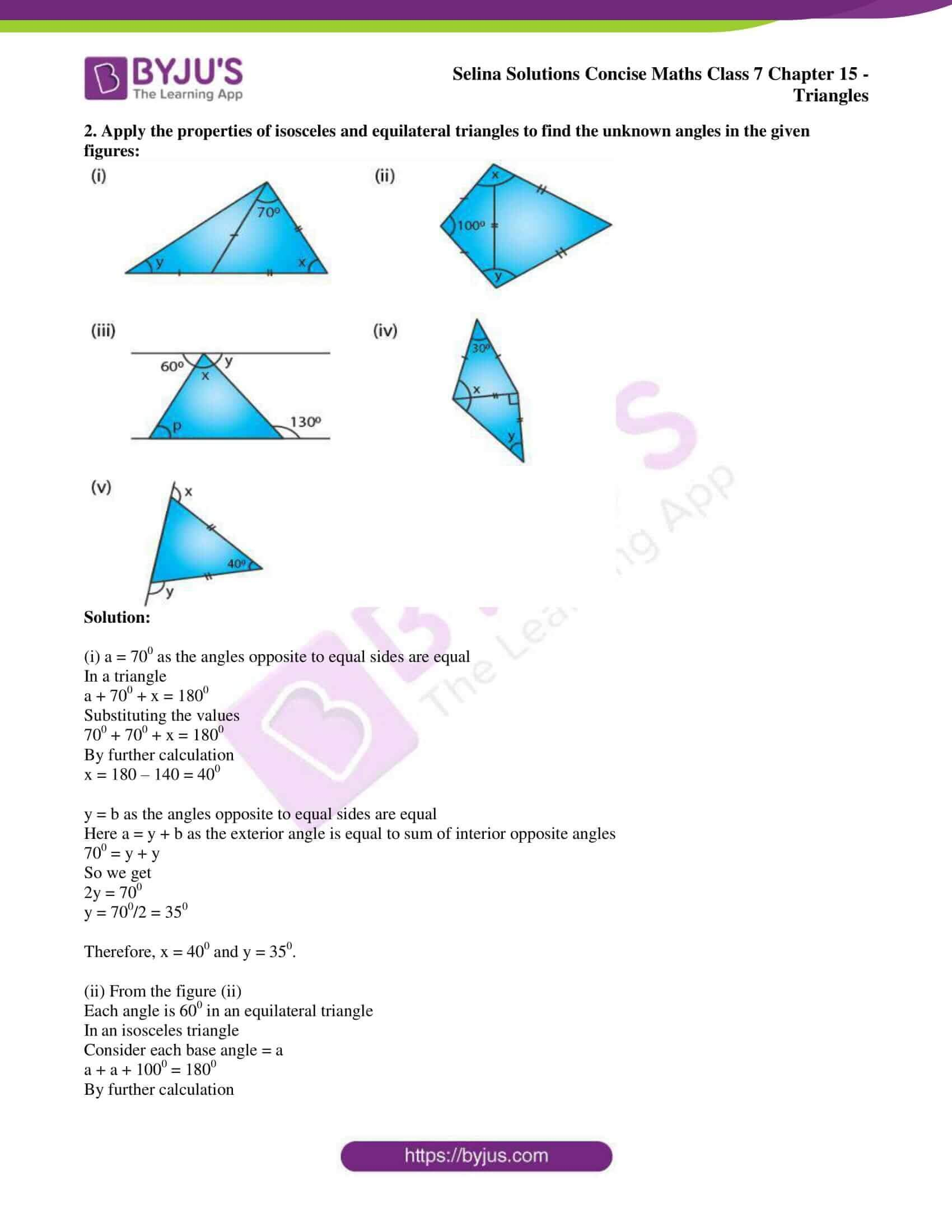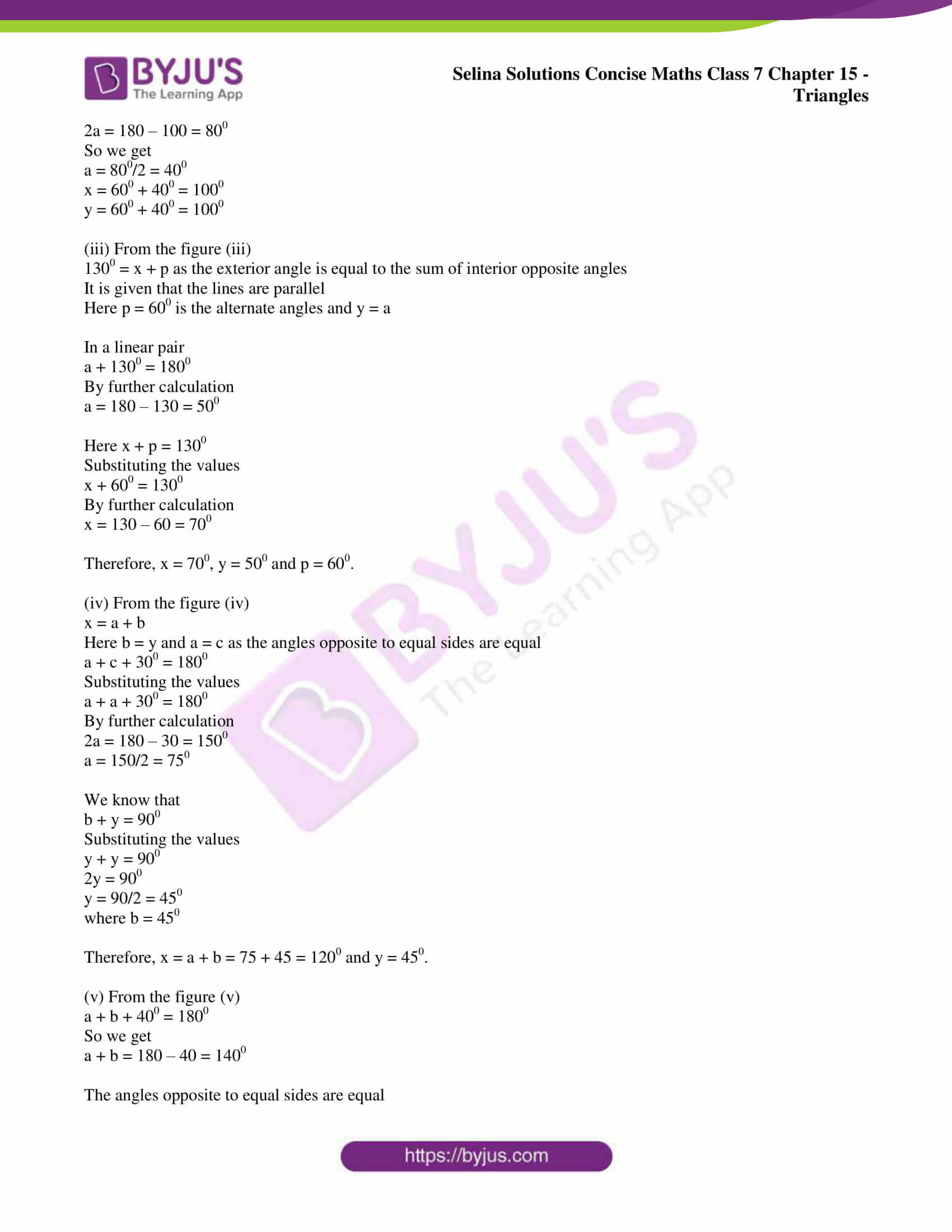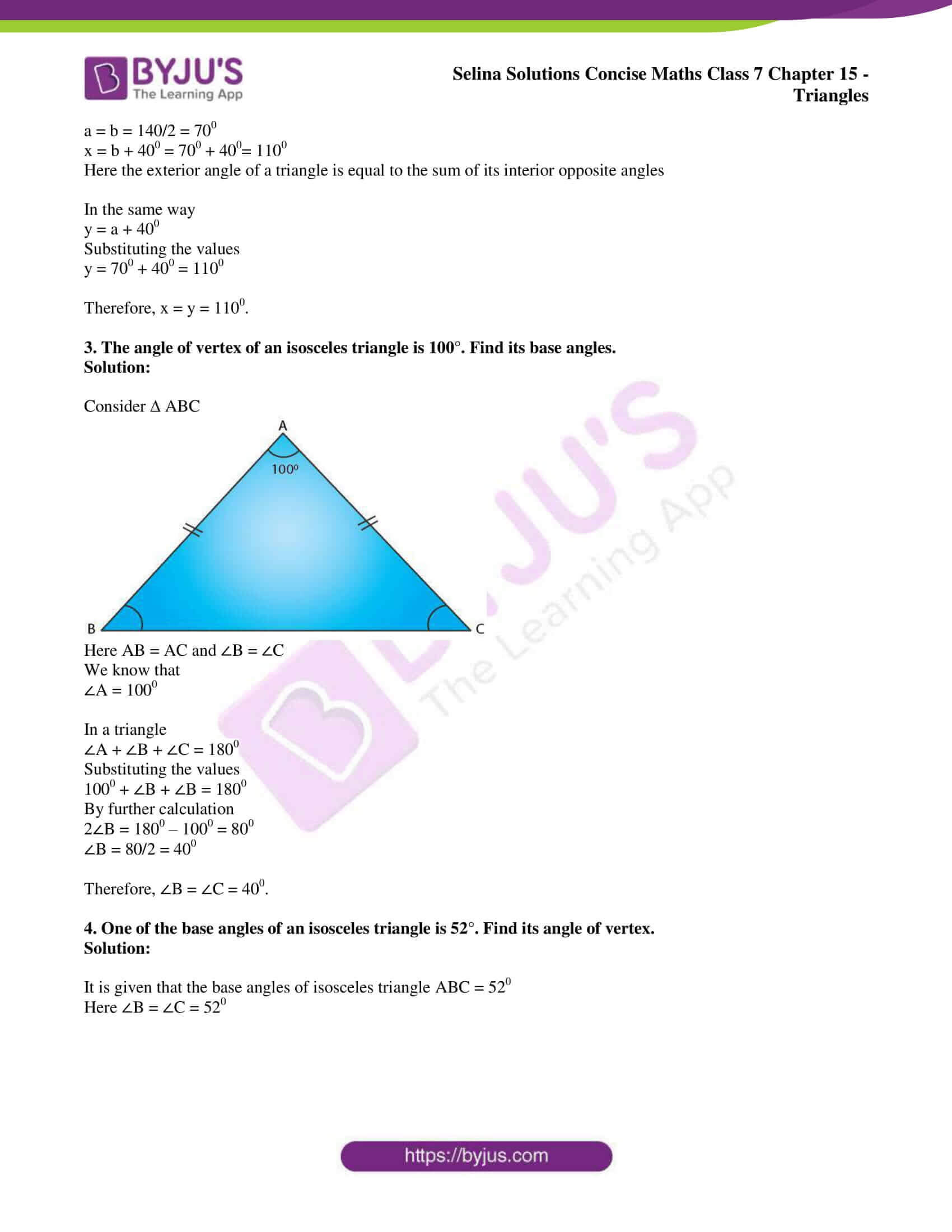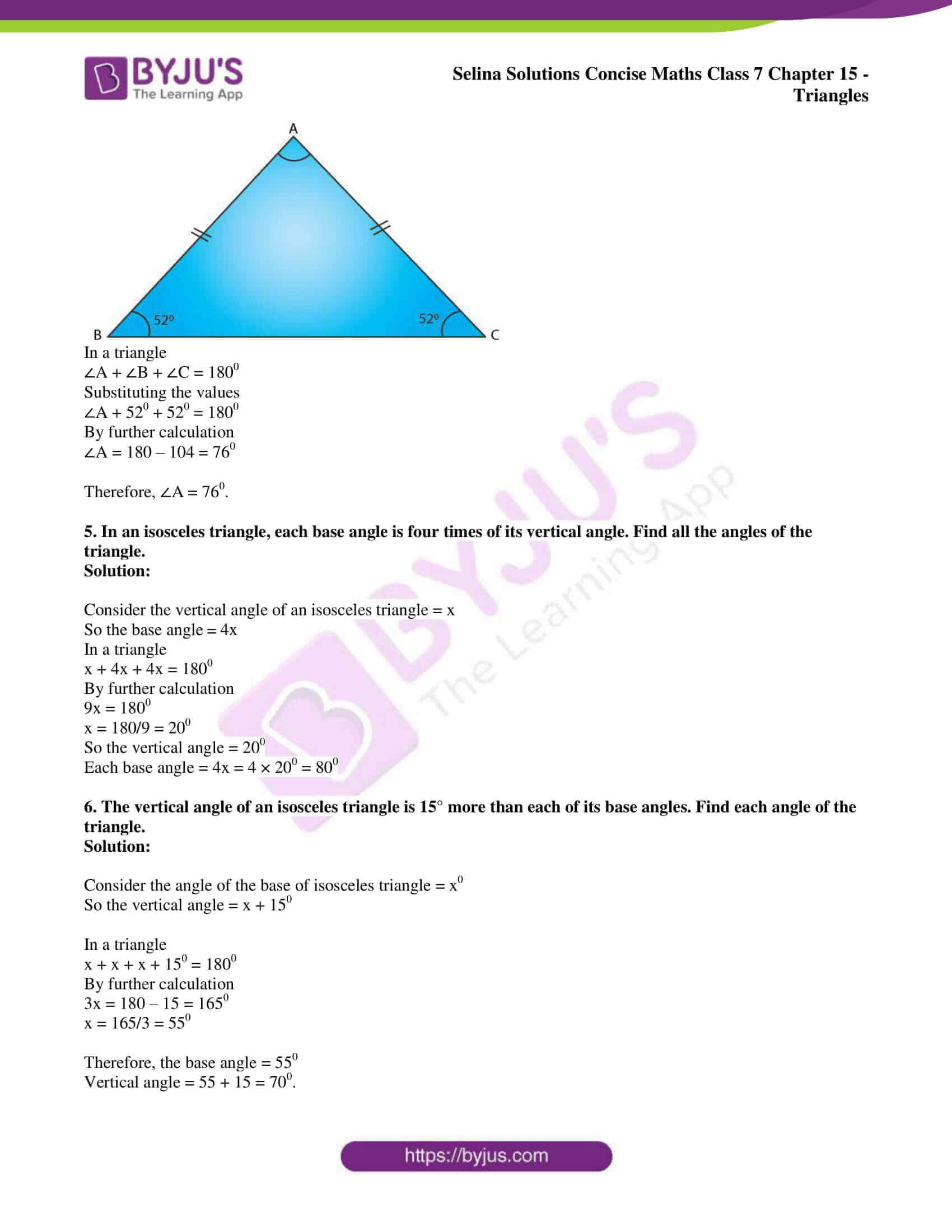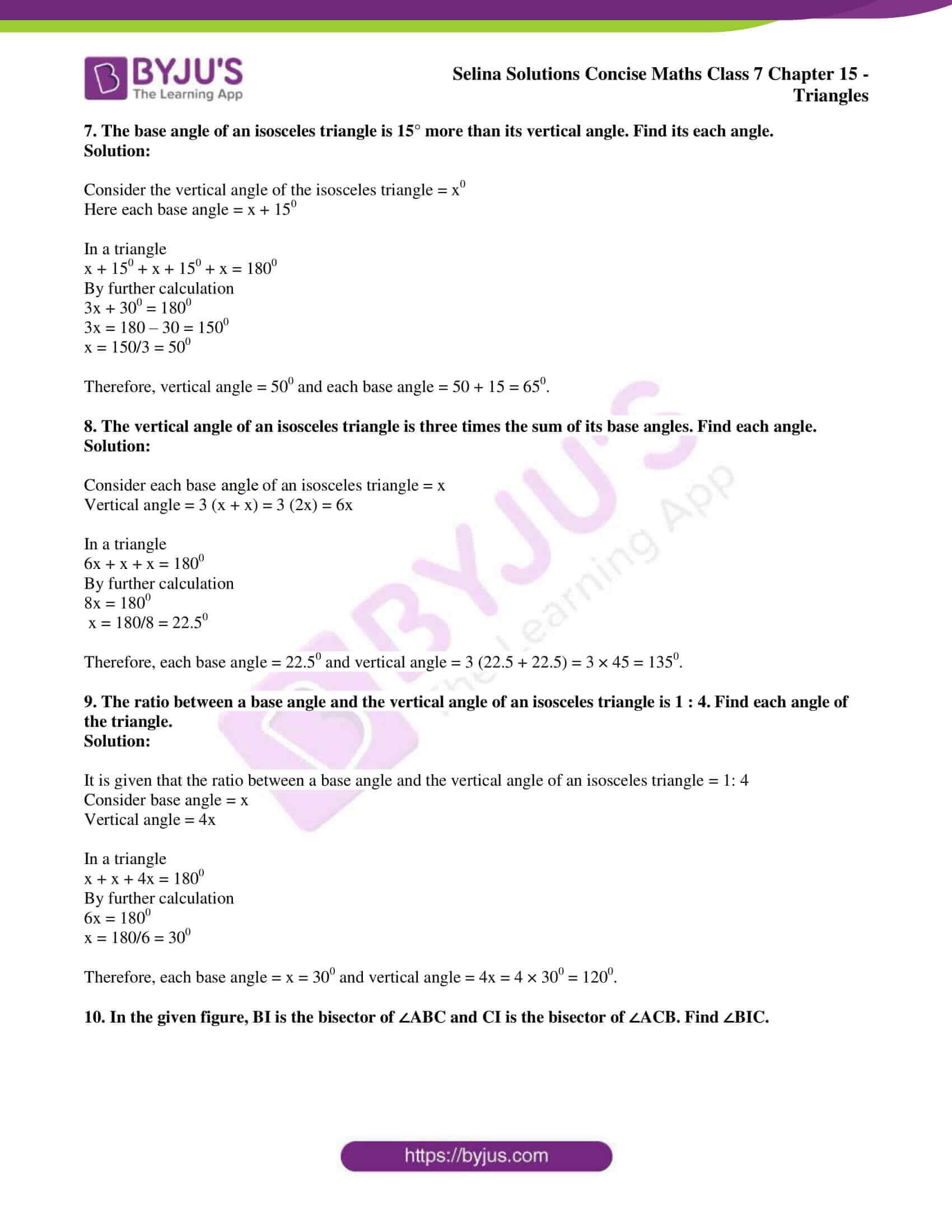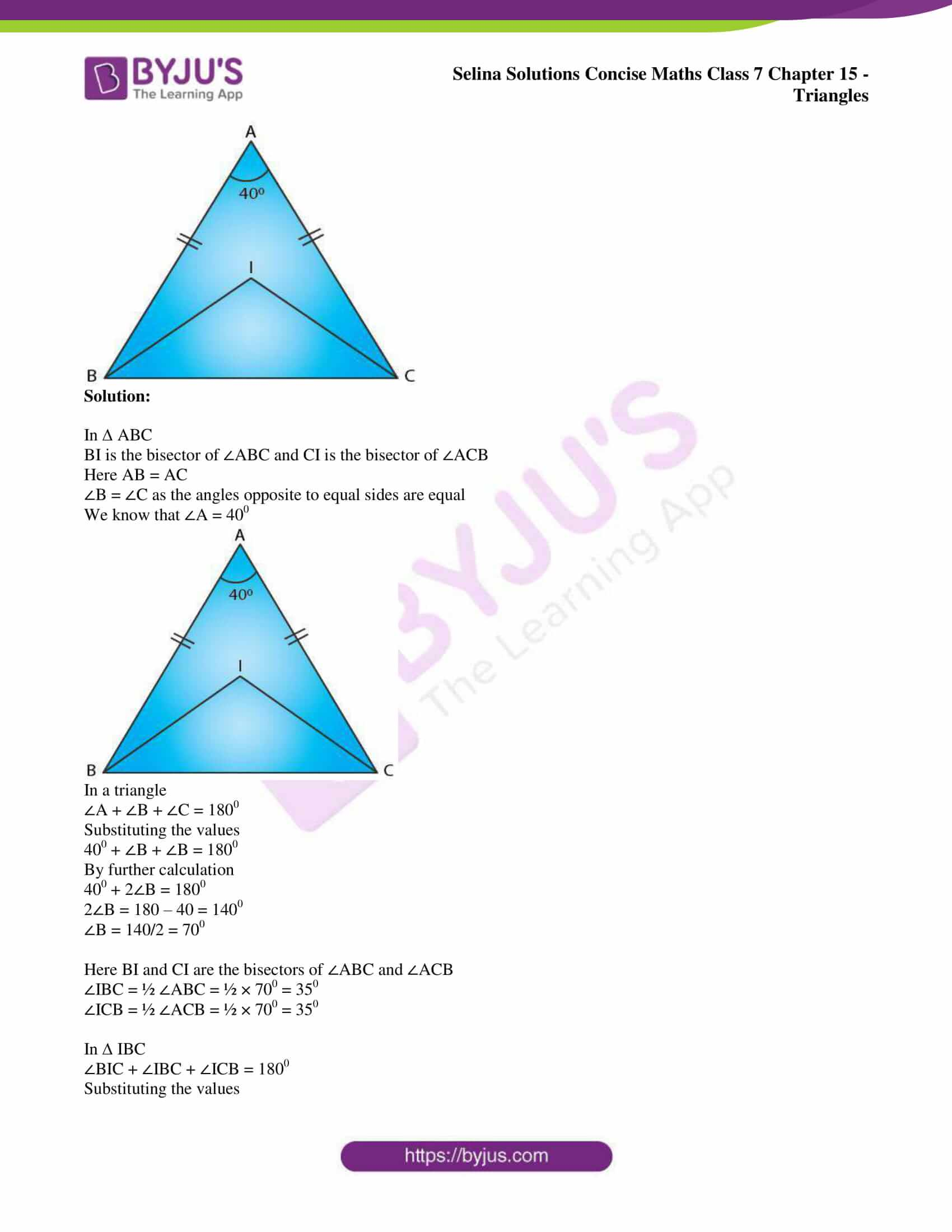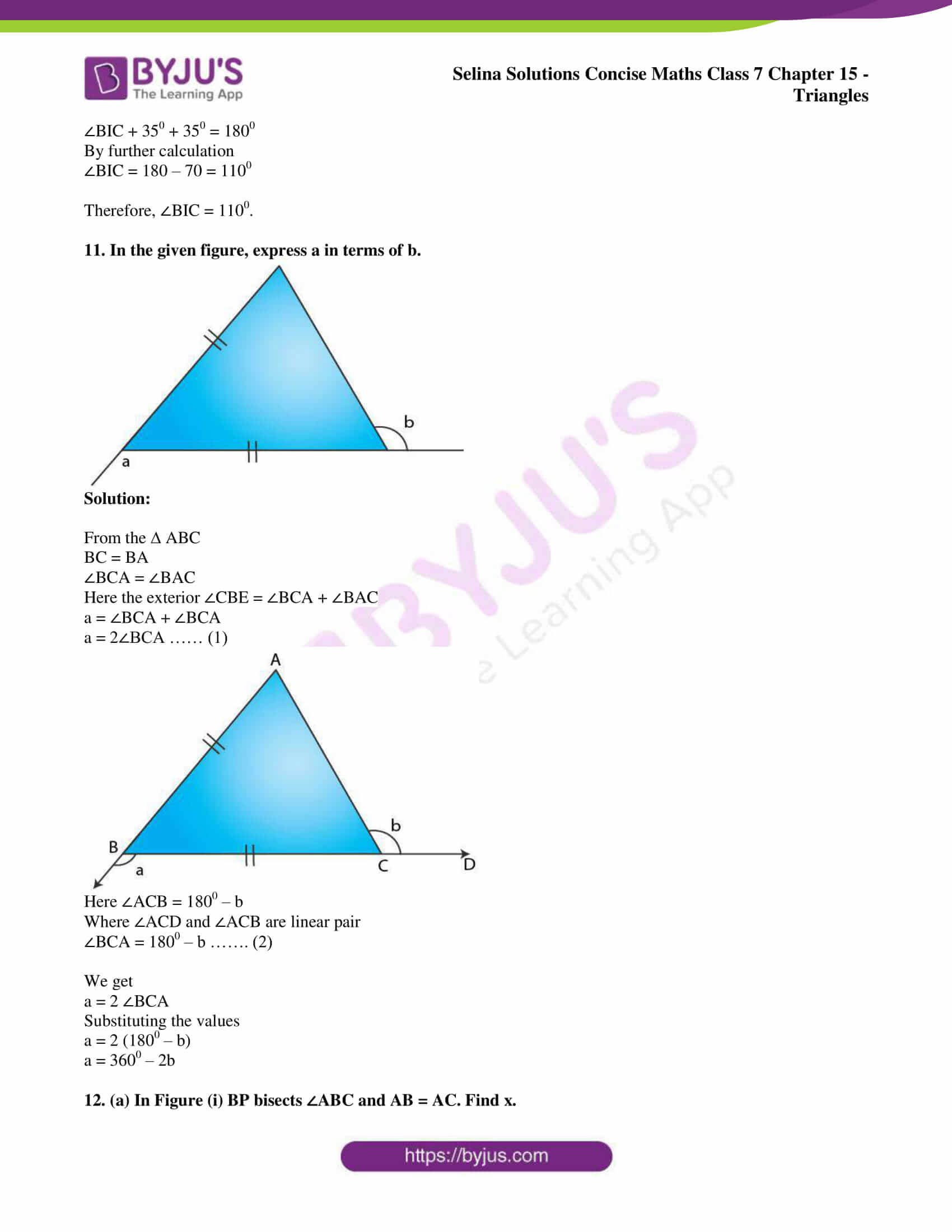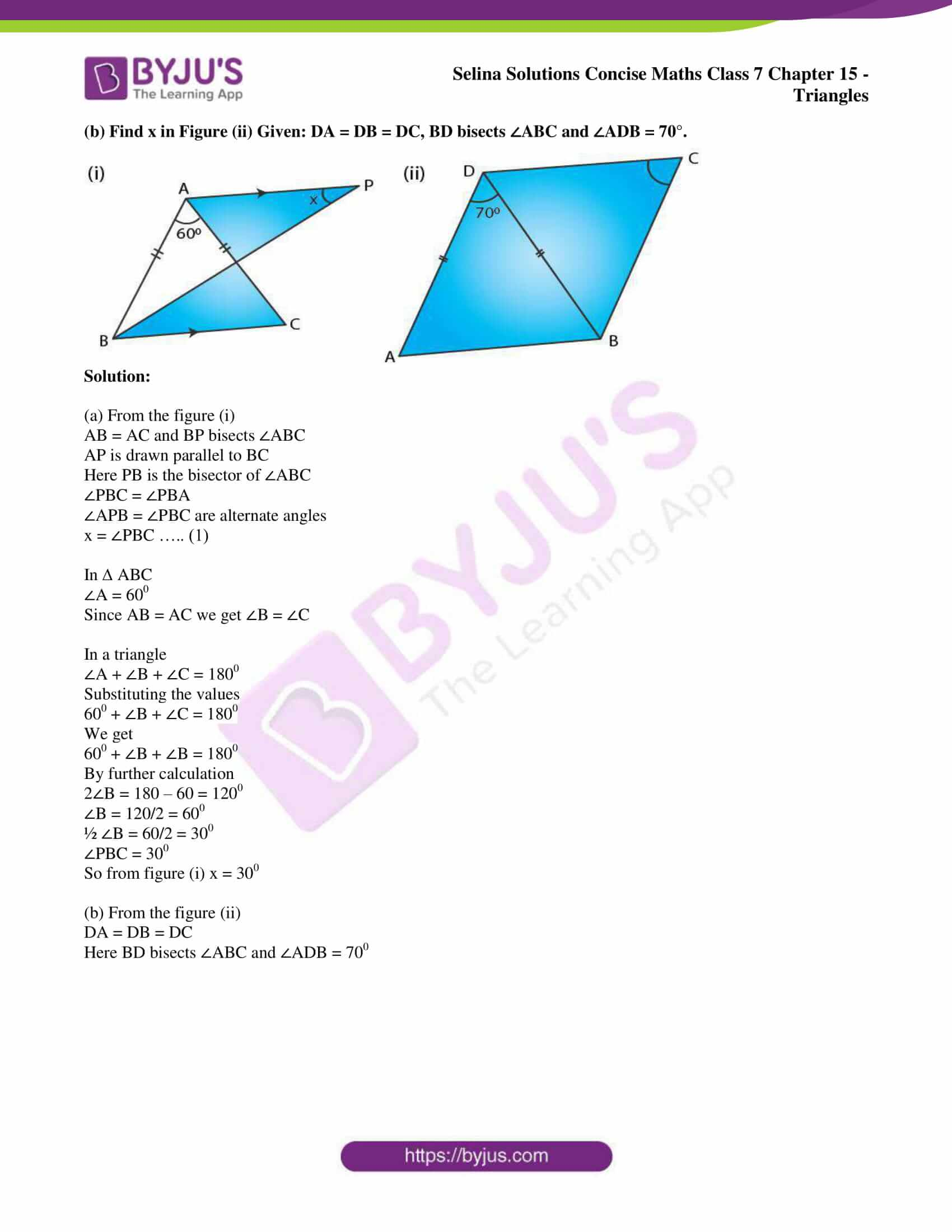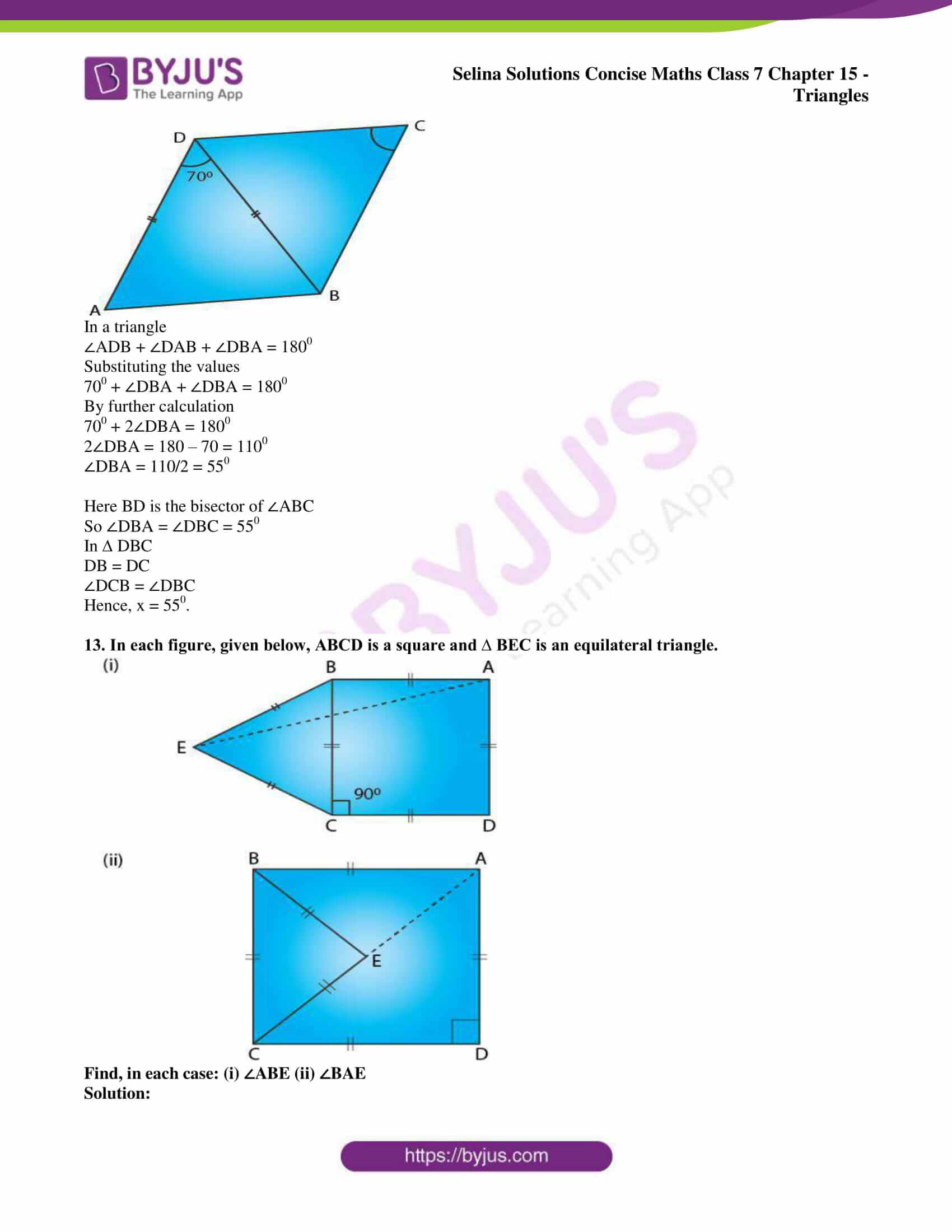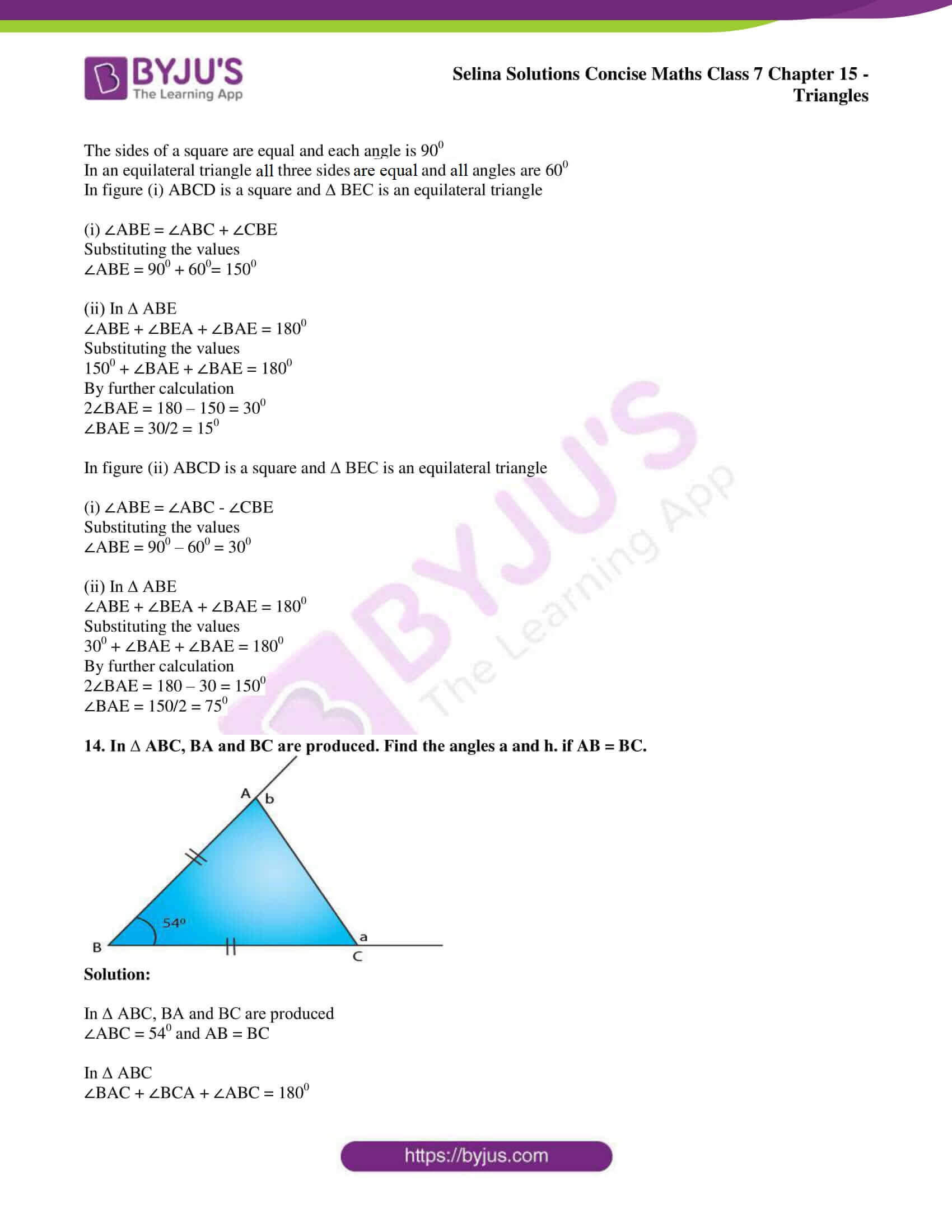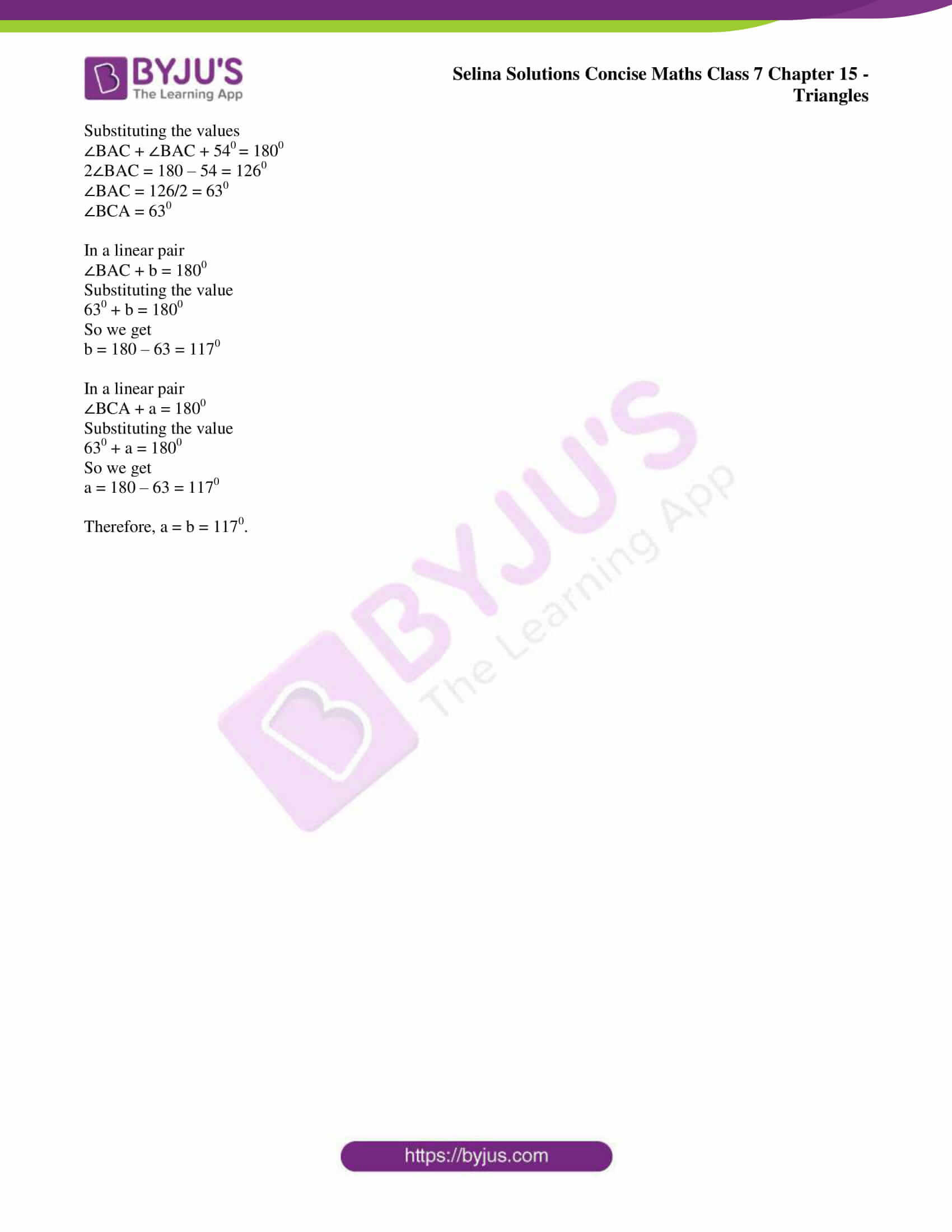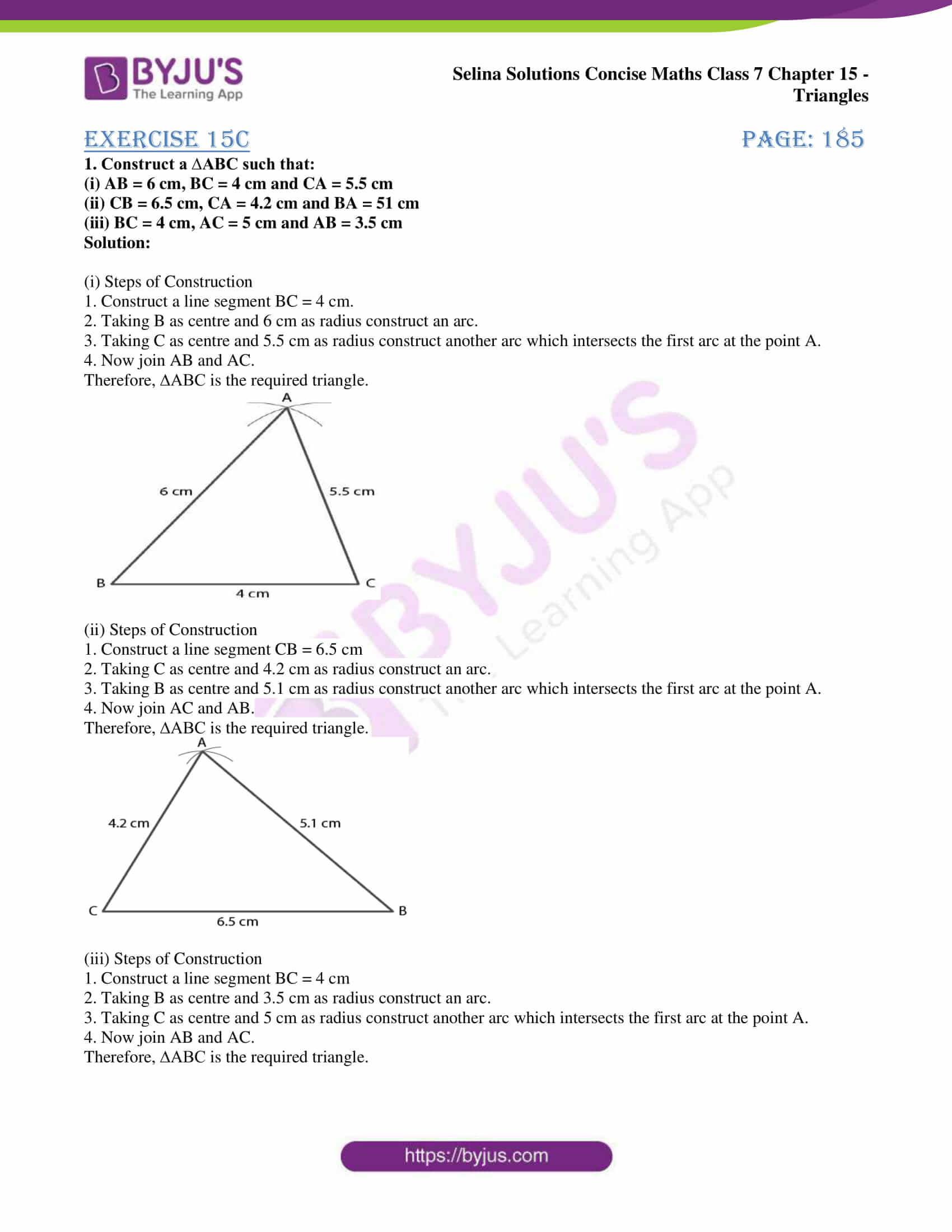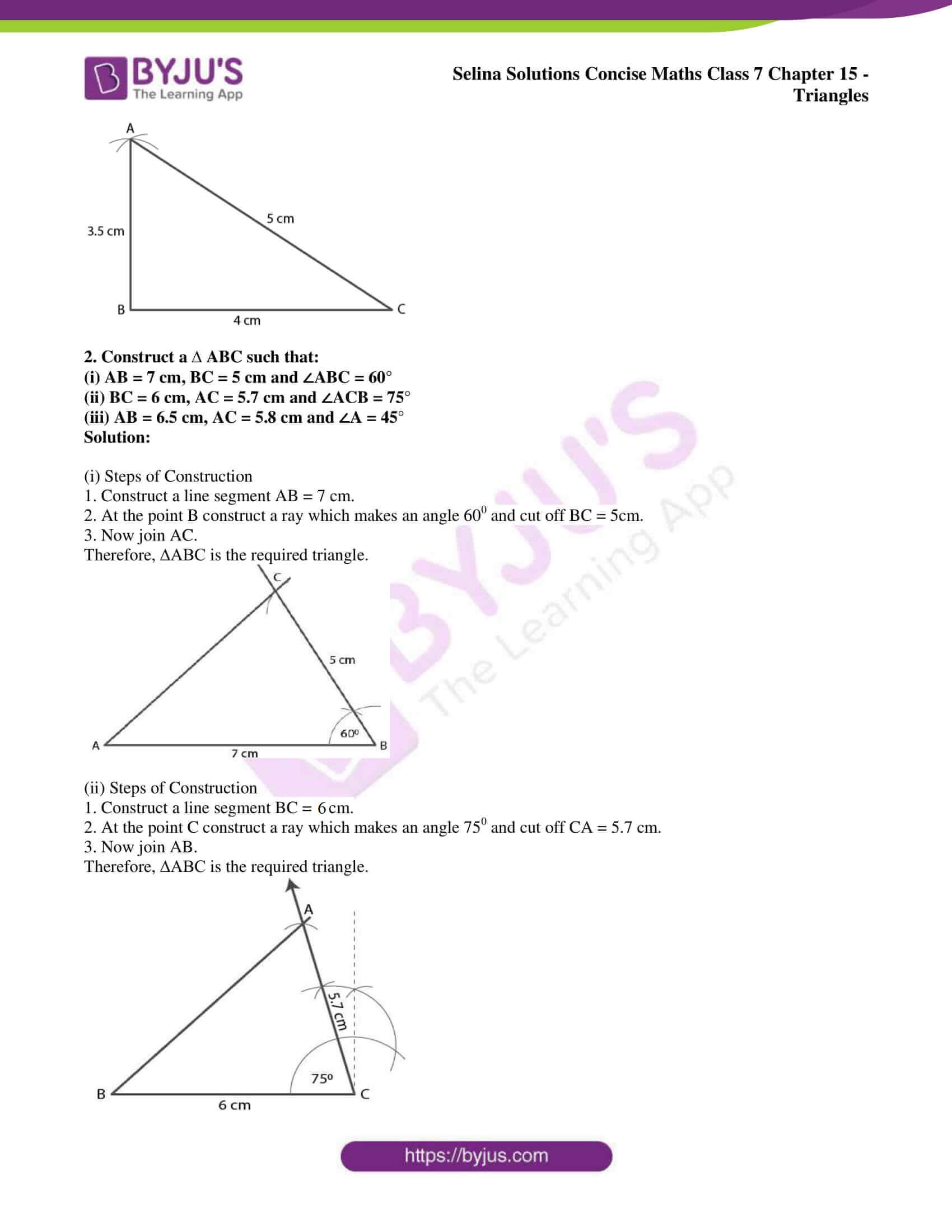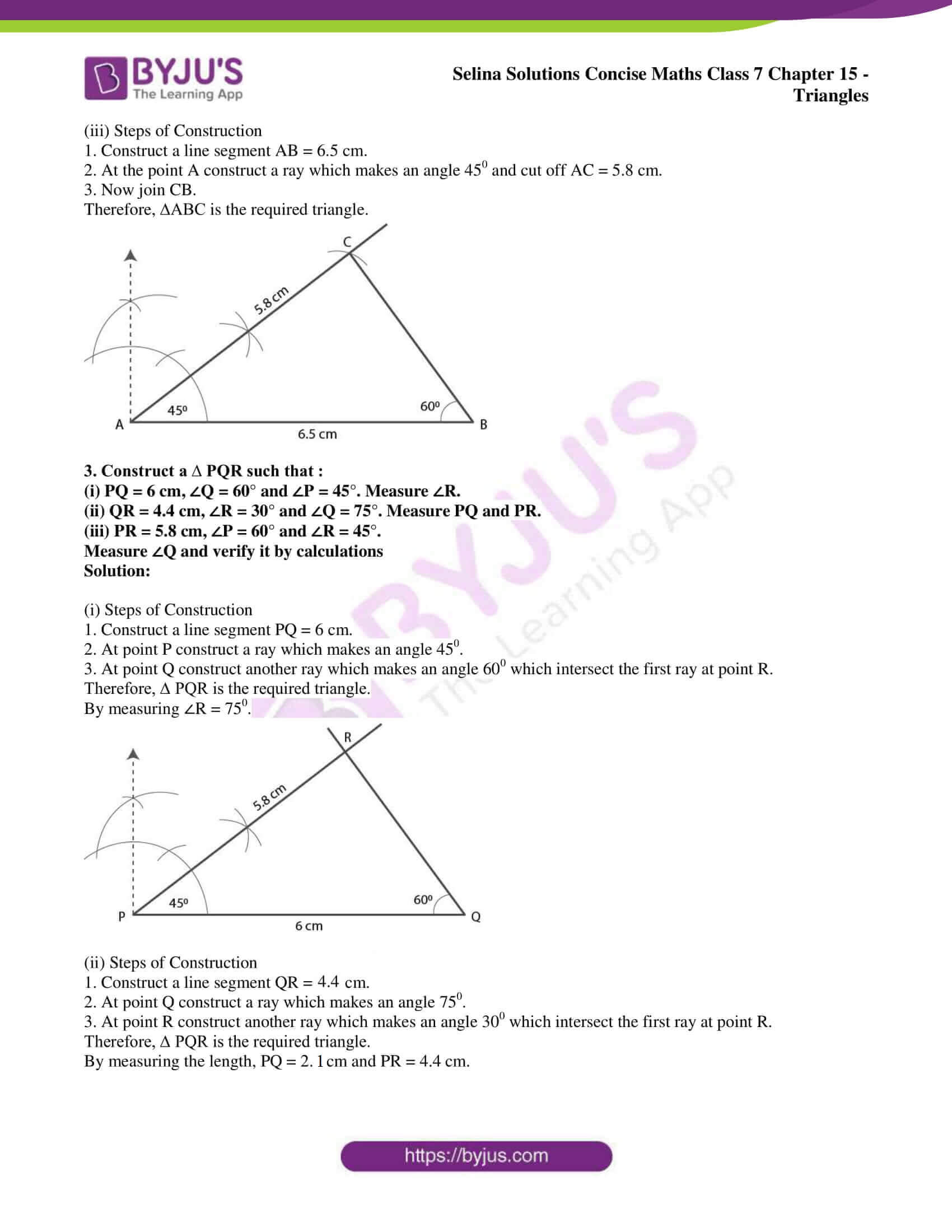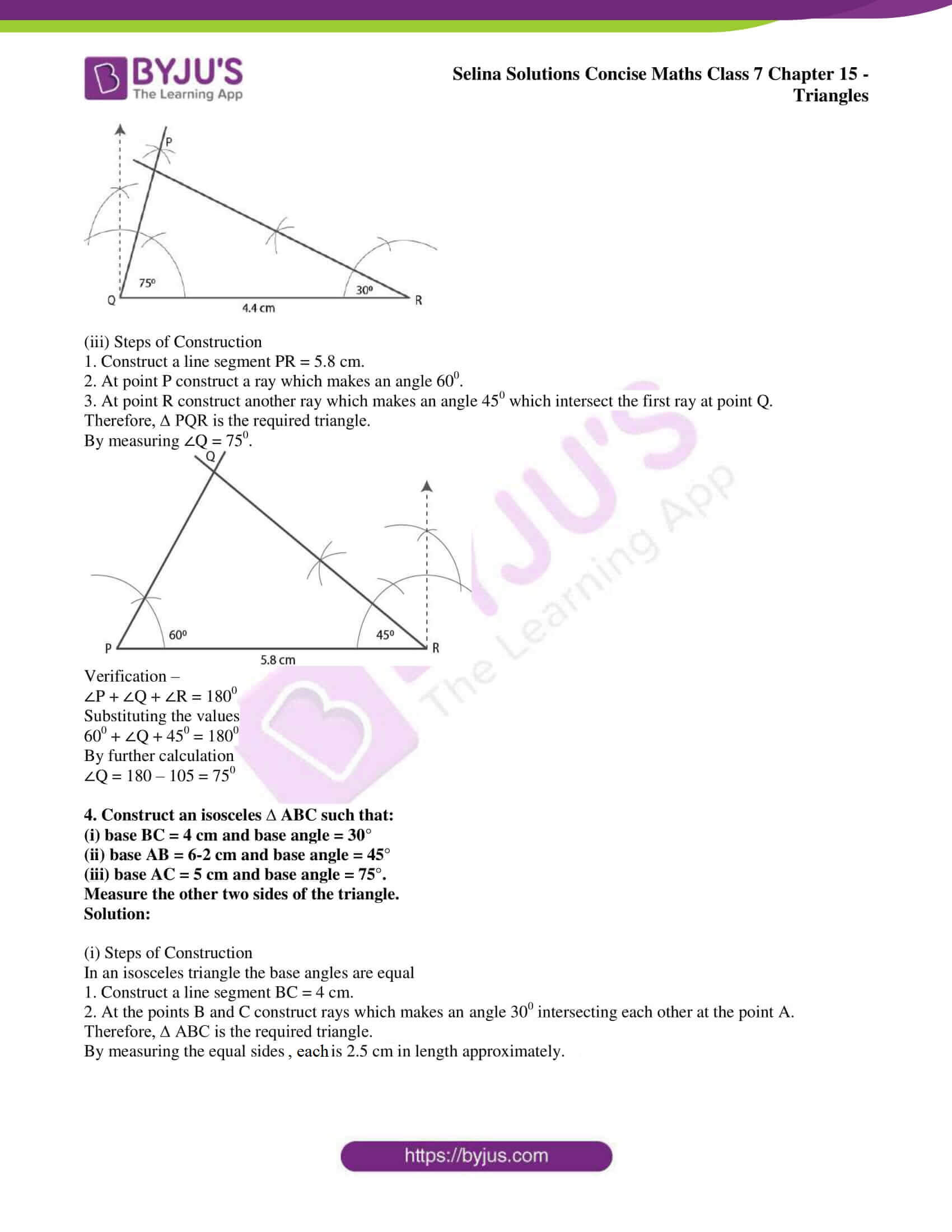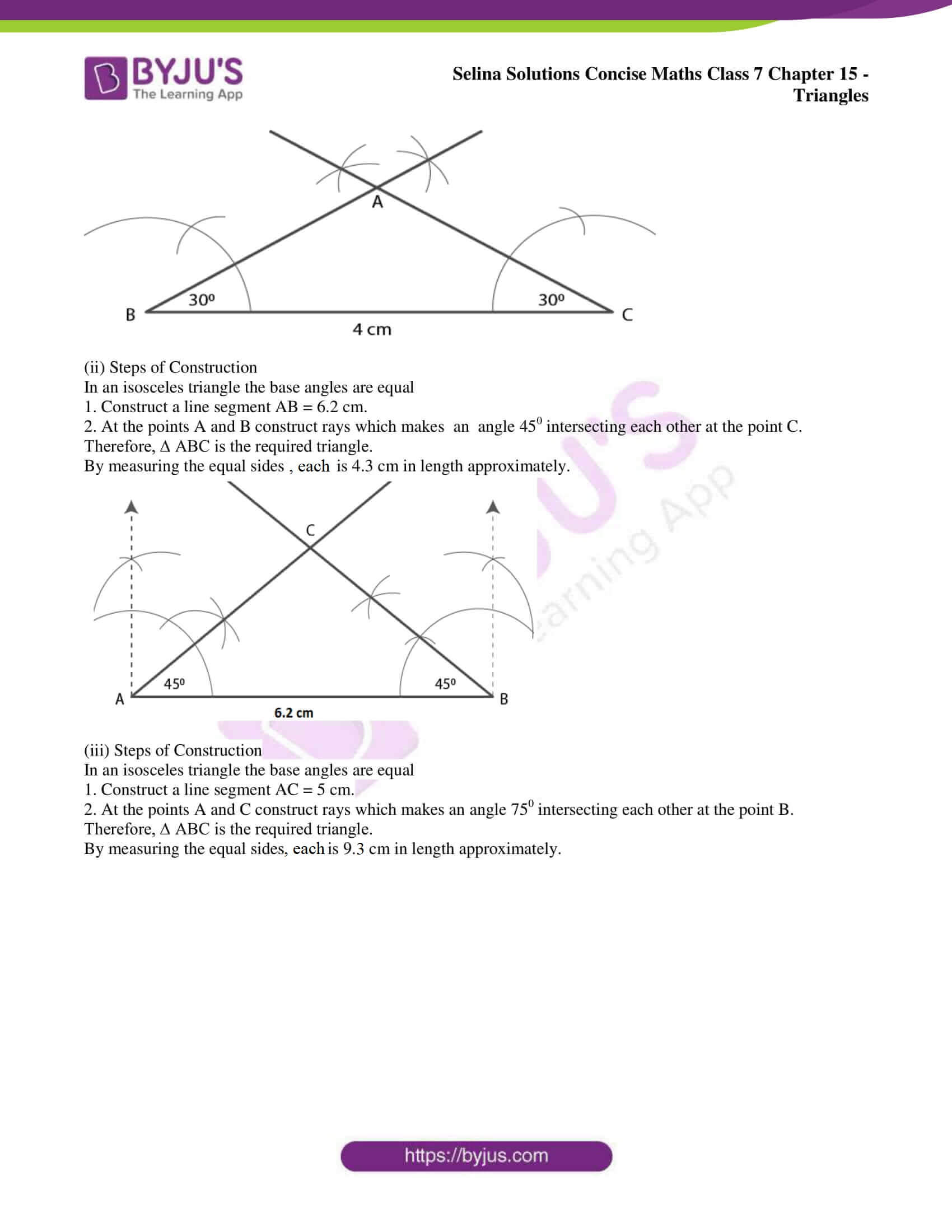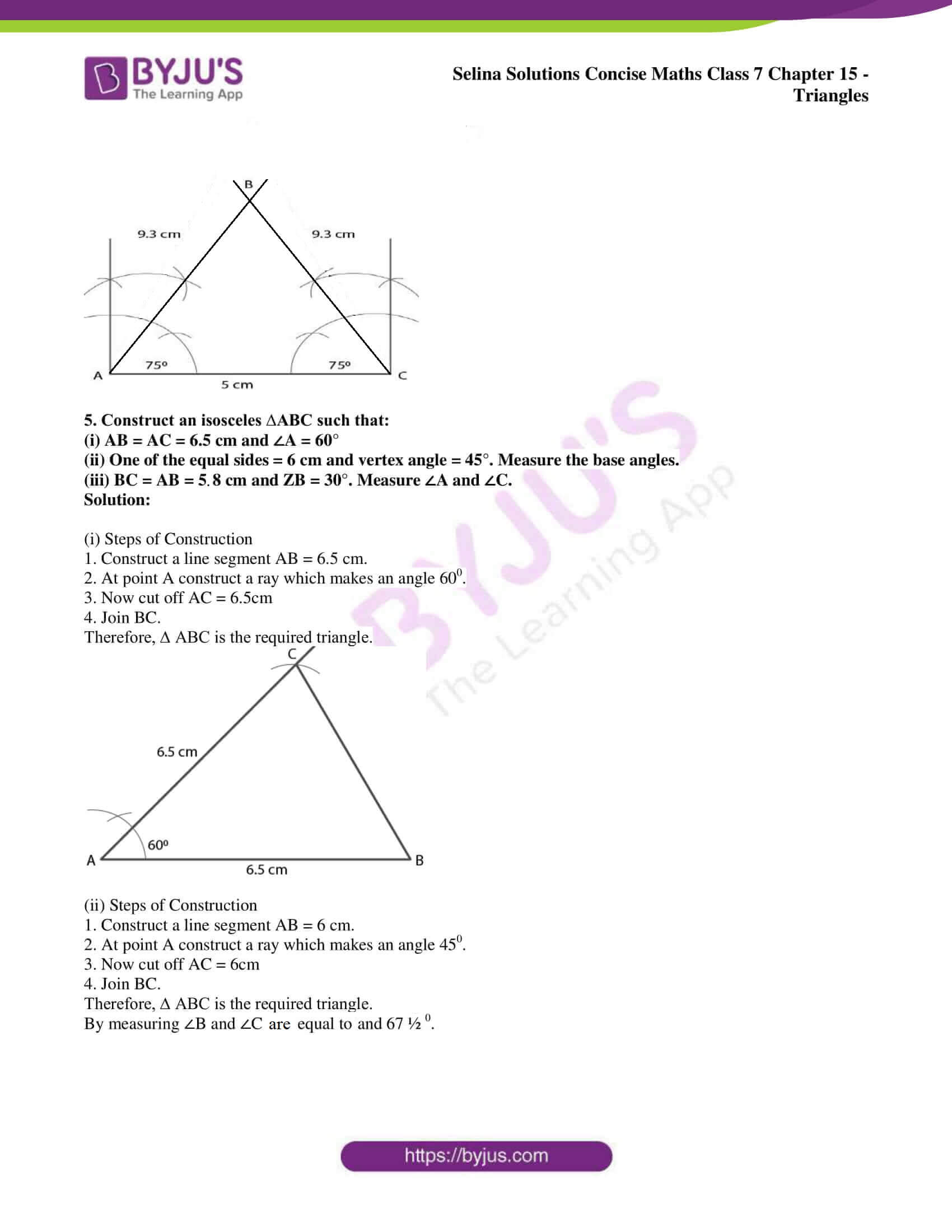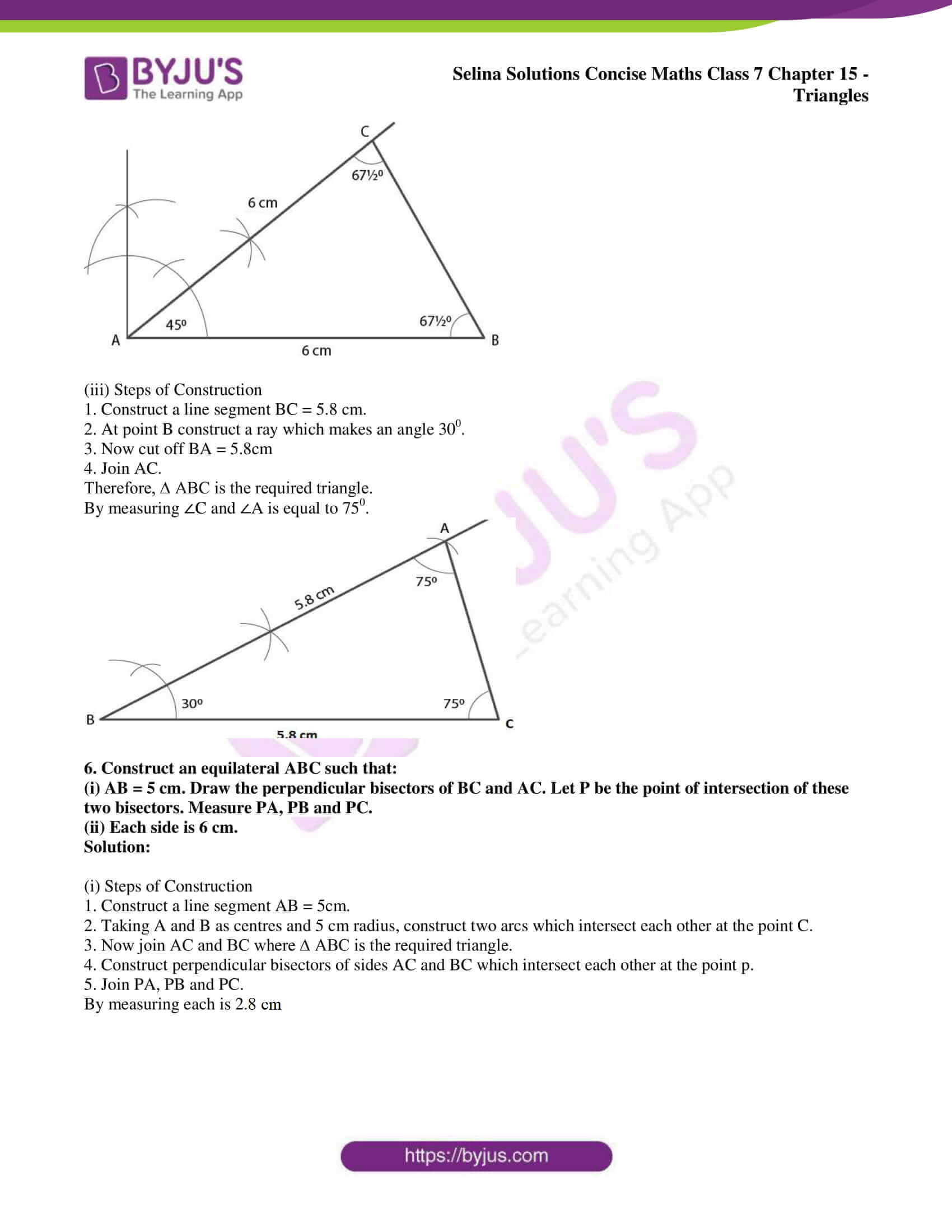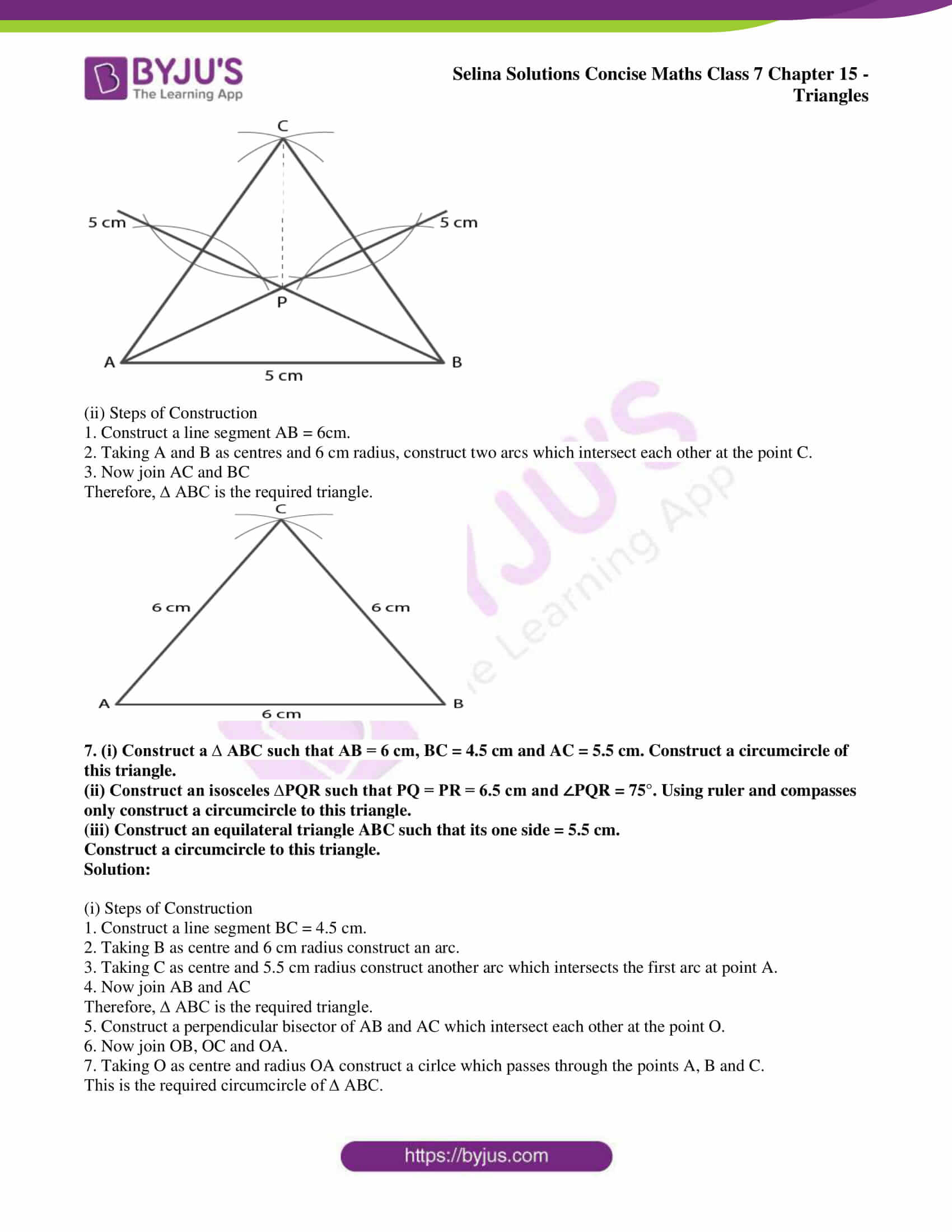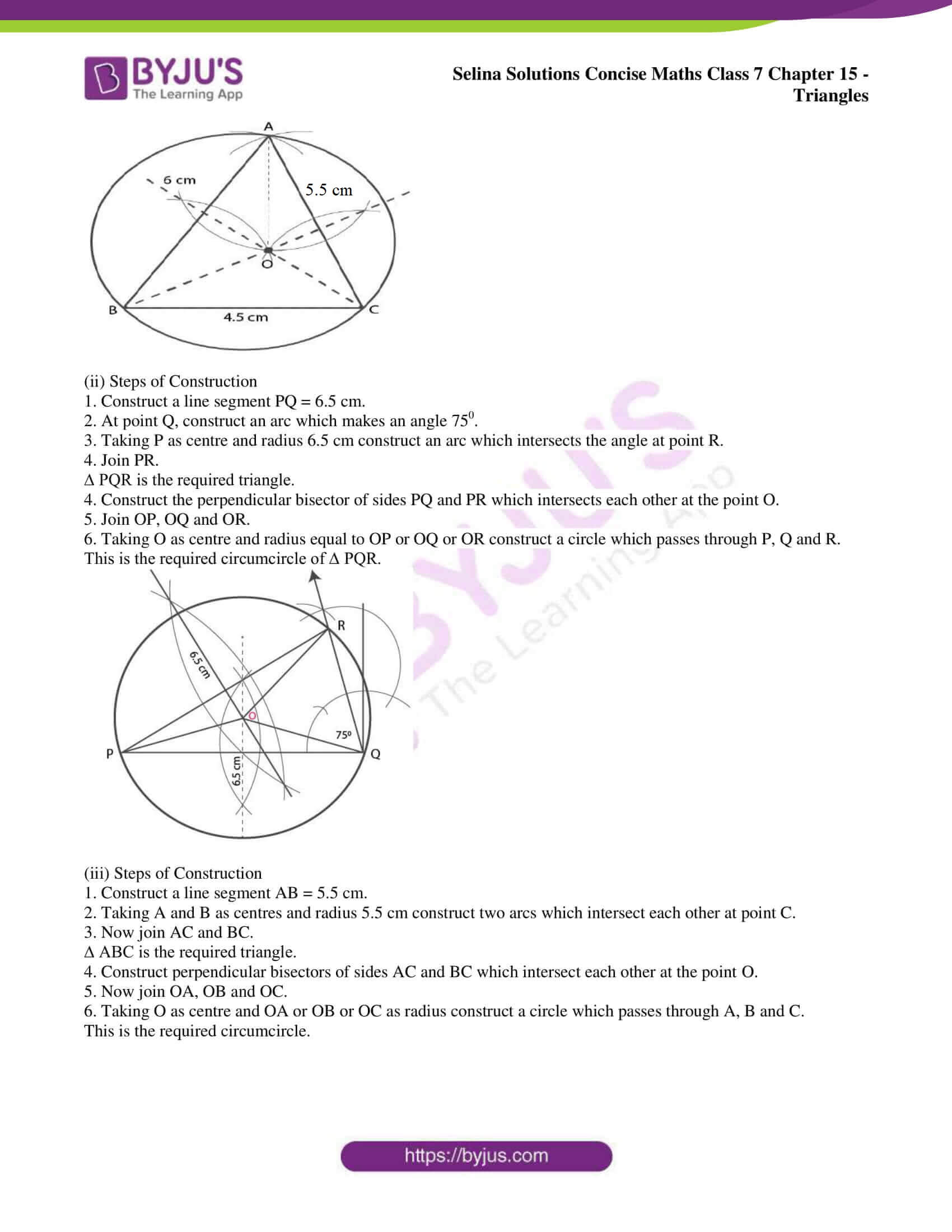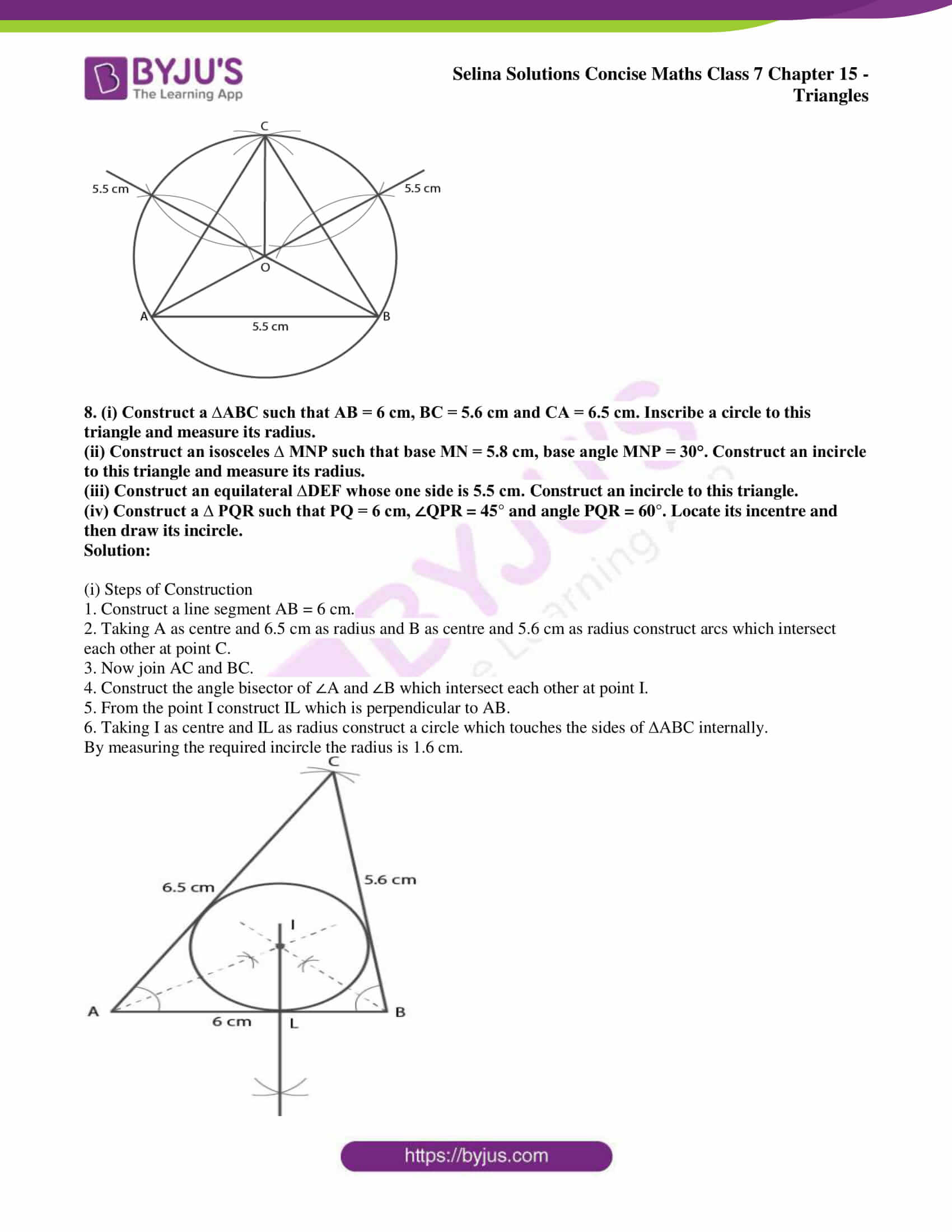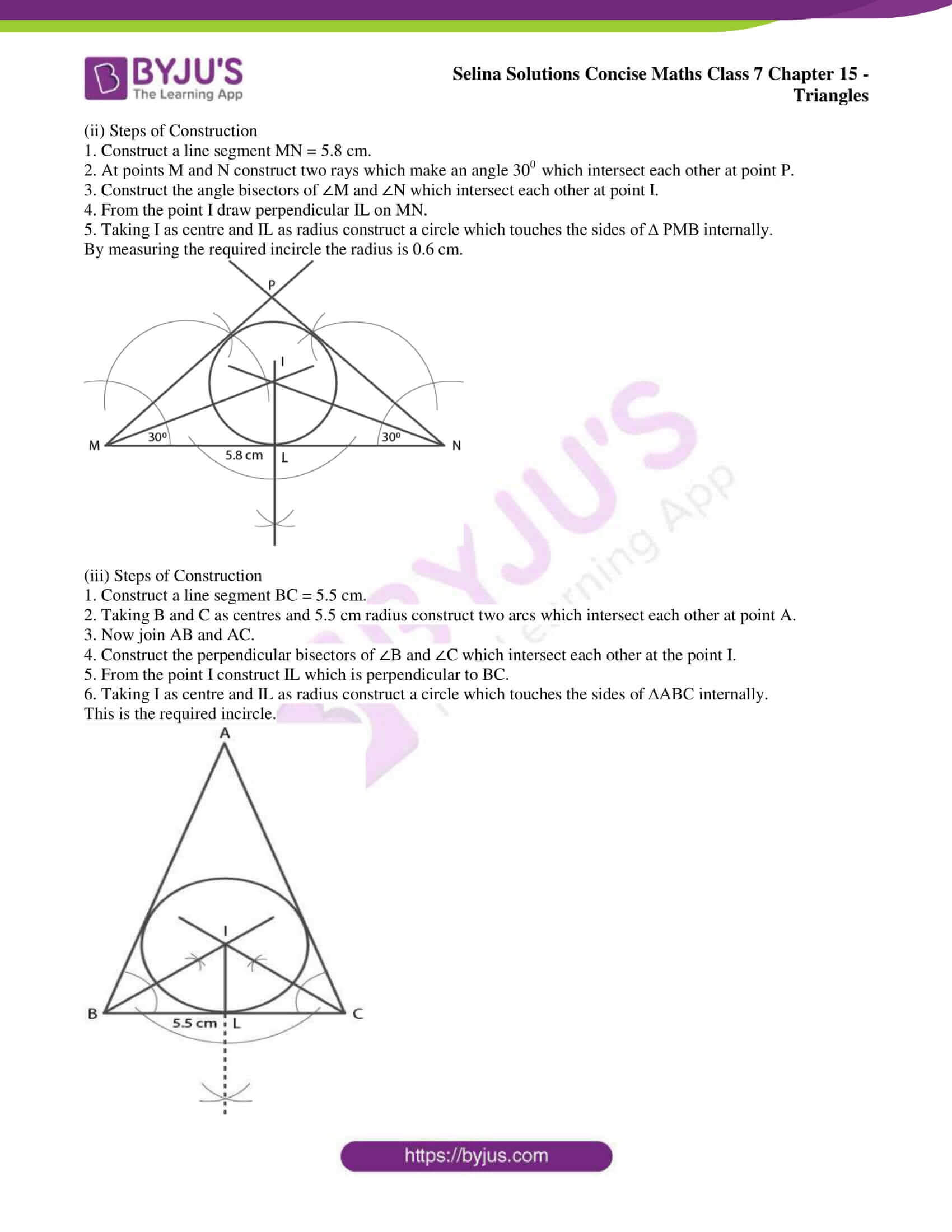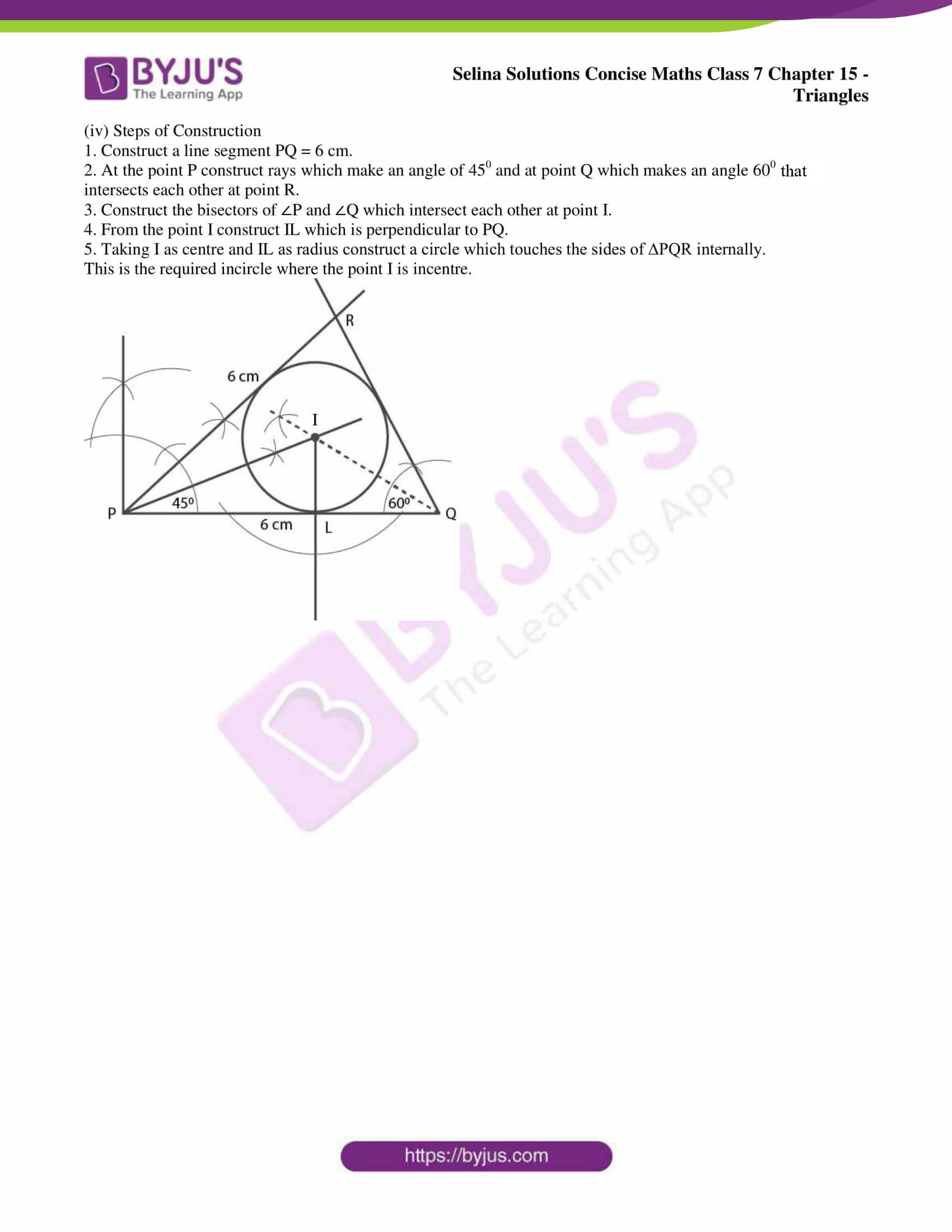### Exercises of Selina Solutions Concise Maths Class 7 Chapter 15 – Triangles

Exercise 15A Solutions

Exercise 15B Solutions

Exercise 15C Solutions

## Access Selina Solutions Concise Maths Class 7 Chapter 15: Triangles

#### Exercise 15A page: 176

1. State, if the triangles are possible with the following angles :
(i) 20°, 70° and 90°
(ii) 40°, 130° and 20°
(iii) 60°, 60° and 50°
(iv) 125°, 40° and 15°

Solution:

In a triangle, the sum of three angles is 1800

(i) 20°, 70° and 90°

Sum = 200 + 700 + 900 = 1800

Here the sum is 1800 and therefore it is possible.

(ii) 40°, 130° and 20°

Sum = 40° + 130° + 20° = 1900

Here the sum is not 1800 and therefore it is not possible.

(iii) 60°, 60° and 50°

Sum = 60° + 60° + 50° = 1700

Here the sum is not 1800 and therefore it is not possible.

(iv) 125°, 40° and 15°

Sum = 125° + 40° + 15° = 1800

Here the sum is 1800 and therefore it is possible.

2. If the angles of a triangle are equal, find its angles.

Solution:

In a triangle, the sum of three angles is 1800

So each angle = 1800/3 = 600

3. In a triangle ABC, ∠A = 45° and ∠B = 75°, find ∠C.

Solution:

In a triangle, the sum of three angles is 1800

∠A + ∠B + ∠C = 1800

Substituting the values

450 + 750 + ∠C = 1800

By further calculation

1200 + ∠C = 1800

So we get

∠C = 1800 – 1200 = 600

4. In a triangle PQR, ∠P = 60° and ∠Q = ∠R, find ∠R.

Solution:

Consider ∠Q = ∠R = x

∠P = 60°

We can write it as

∠P + ∠Q + ∠R = 1800

Substituting the values

600 + x + x = 1800

By further calculation

600 + 2x = 1800

2x = 1800 – 600 = 1200

So we get

x = 1200/2 = 600

∠Q = ∠R = 600

Therefore, ∠R = 600.

5. Calculate the unknown marked angles in each figure: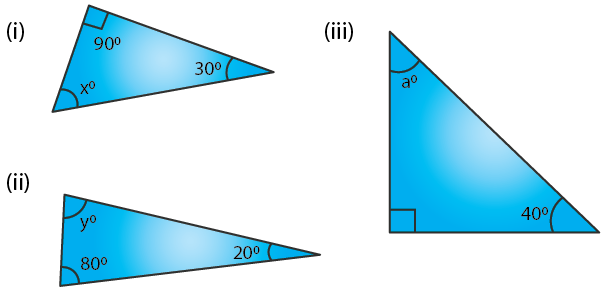Solution:

In a triangle, the sum of three angles is 1800

(i) From figure (i)

900 + 300 + x = 1800

By further calculation

1200 + x = 1800

So we get

x = 1800 – 1200 = 600

Therefore, x = 600.

(ii) From figure (ii)

y + 800 + 200 = 1800

By further calculation

y + 1000 = 1800

So we get

y = 1800 – 1000 = 800

Therefore, y = 800.

(iii) From figure (iii)

a + 900 + 400 = 1800

By further calculation

a + 1300 = 1800

So we get

a = 1800 – 1300 = 500

Therefore, a = 500.

6. Find the value of each angle in the given figures: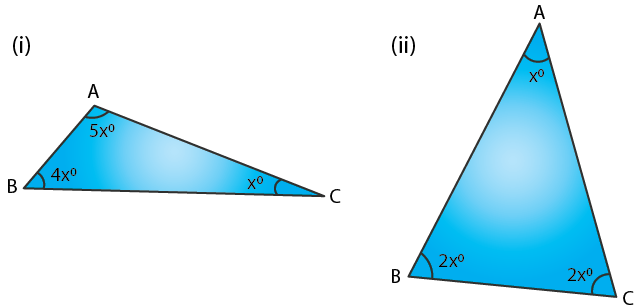Solution:

(i) From the figure (i)

∠A + ∠B + ∠C = 1800

Substituting the values

5x0 + 4x0 + x0 = 1800

By further calculation

10x0 = 1800

x = 180/10 = 180

So we get

∠A = 5x0 = 5 × 180 = 900

∠B = 4x0 = 4 × 180 = 720

∠C = x = 180

(ii) From the figure (ii)

∠A + ∠B + ∠C = 1800

Substituting the values

x0 + 2x0 + 2x0 = 1800

By further calculation

5x0 = 1800

x0 = 1800/5 = 360

So we get

∠A = x0 = 360

∠B = 2x0 = 2 × 360 = 720

∠C = 2x0 = 2 × 360 = 720

7. Find the unknown marked angles in the given figure: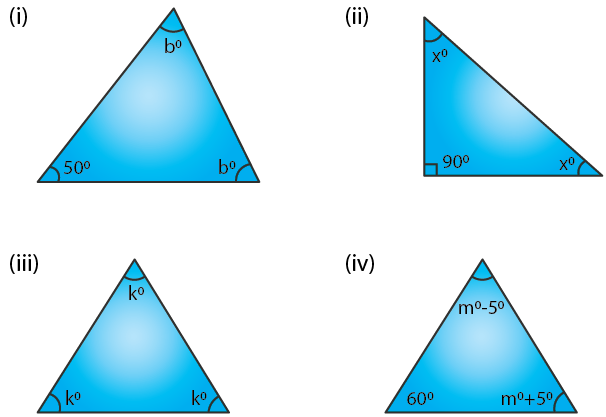Solution:

(i) From the figure (i)

∠A + ∠B + ∠C = 1800

Substituting the values

b0 + 500 + b0 = 1800

By further calculation

2b0 = 1800 – 500 = 1300

b0 = 1300/2 = 650

Therefore, ∠A = ∠C = b0 = 650.

(ii) From the figure (ii)

∠A + ∠B + ∠C = 1800

Substituting the values

x0 + 900 + x0 = 1800

By further calculation

2x0 = 1800 – 900 = 900

x0 = 900/2 = 450

Therefore, ∠A = ∠C = x0 = 450.

(iii) From the figure (iii)

∠A + ∠B + ∠C = 1800

Substituting the values

k0 + k0 + k0 = 1800

By further calculation

3k0 = 1800

k0 = 1800/3 = 600

Therefore, ∠A = ∠B = ∠C = 600.

(iv) From the figure (iv)

∠A + ∠B + ∠C = 1800

Substituting the values

(m0 – 50) + 600 + (m0 + 50) = 1800

By further calculation

m0 – 50 + 600 + m0 + 50 = 1800

2m0 = 1800 – 600 = 1200

m0 = 1200/2 = 600

Therefore, ∠A = m0 – 50 = 600 – 50 = 550

∠C = m0 + 50 = 600 + 50 = 650

8. In the given figure, show that: ∠a = ∠b + ∠c

(i) If ∠b = 60° and ∠c = 50°; find ∠a.
(ii) If ∠a = 100° and ∠b = 55°; find ∠c.
(iii) If ∠a = 108° and ∠c = 48°; find ∠b.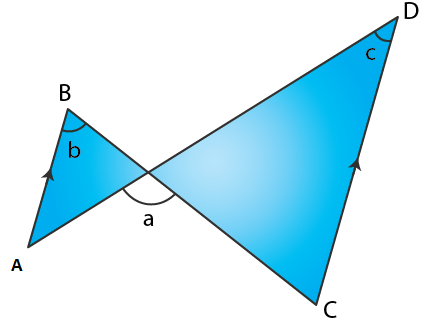Solution:

From the figure

AB || CD

b = ∠C and ∠A = c are alternate angles

In triangle PCD

Exterior ∠APC = ∠C + ∠D

a = b + c

(i) If ∠b = 60° and ∠c = 50°

∠a = ∠b + ∠c

Substituting the values

∠a = 60 + 50 = 1100

(ii) If ∠a = 100° and ∠b = 55°

∠a = ∠b + ∠c

Substituting the values

∠c = 100 – 55 = 450

(iii) If ∠a = 108° and ∠c = 48°

∠a = ∠b + ∠c

Substituting the values

∠b = 108 – 48 = 600

9. Calculate the angles of a triangle if they are in the ratio 4 : 5 : 6.

Solution:

In a triangle, the sum of angles of a triangle is 1800

∠A + ∠B + ∠C = 1800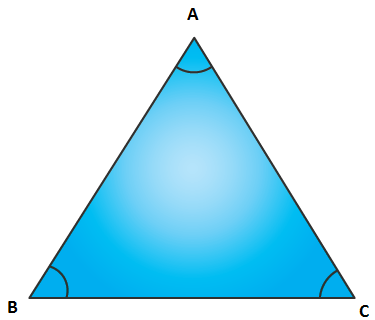It is given that

∠A: ∠B: ∠C = 4: 5: 6

Consider ∠A = 4x, ∠B = 5x and ∠C = 6x

Substituting the values

4x + 5x + 6x = 1800

By further calculation

15x = 1800

x = 1800/15 = 120

So we get

∠A = 4x = 4 × 120 = 480

∠B = 5x = 5 × 120 = 600

∠C = 6x = 6 × 120 = 720

10. One angle of a triangle is 60°. The, other two angles are in the ratio of 5 : 7. Find the two angles.

Solution:

From the triangle ABC

Consider ∠A = 600, ∠B: ∠C = 5:7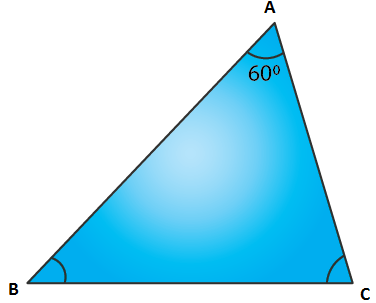In a triangle

∠A + ∠B + ∠C = 1800

Substituting the values

600 + ∠B + ∠C = 1800

By further calculation

∠B + ∠C = 1800 – 600 = 1200

Take ∠B = 5x and ∠C = 7x

Substituting the values

5x + 7x = 1200

12x = 1200

x = 1200/12 = 100

So we get

∠B = 5x = 5 × 100 = 500

∠C = 7x = 7 × 100 = 700

11. One angle of a triangle is 61° and the other two angles are in the ratio 1 ½ : 1 1/3. Find these angles.

Solution:

From the triangle ABC

Consider ∠A = 610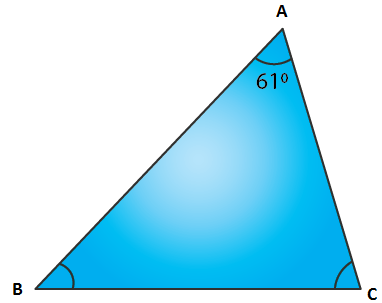In a triangle

∠A + ∠B + ∠C = 1800

Substituting the values

610 + ∠B + ∠C = 1800

By further calculation

∠B + ∠C = 1800 – 610 = 1190

∠B: ∠C = 1 ½: 1 1/3 = 3/2: 4/3

Taking LCM

∠B: ∠C = 9/6: 8/ 6

∠B: ∠C = 9: 8

Consider ∠B = 9x and ∠C = 8x

Substituting the values

9x + 8x = 1190

17x = 1190

x = 1190/ 17 = 70

So we get

∠B = 9x = 9 × 70 = 630

∠C = 8x = 8 × 70 = 560

12. Find the unknown marked angles in the given figures: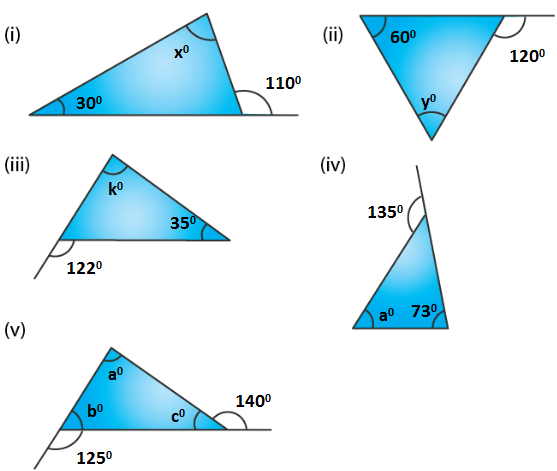Solution:

In a triangle, if one side is produced

Exterior angle is the sum of opposite interior angles

(i) From the figure (i)

1100 = x0 + 300

By further calculation

x0 = 1100 – 300 = 800

(ii) From the figure (ii)

1200 = y0 + 600

By further calculation

y0 = 1200 – 600 = 600

(iii) From the figure (iii)

1220 = k0 + 350

By further calculation

k0 = 1220 – 350 = 870

(iv) From the figure (iv)

1350 = a0 + 730

By further calculation

a0 = 1350 – 730 = 620

(v) From the figure (v)

1250 = a + c …… (1)

1400 = a + b …… (2)

a + c + a + b = 1250 + 1400

On further calculation

a + a + b + c = 2650

We know that a + b + c = 1800

Substituting it in the equation

a + 1800 = 2650

So we get

a = 265 – 180 = 850

If a + b = 1400

Substituting it in the equation

850 + b = 1400

So we get

b = 140 – 85 = 550

If a + c = 1250

Substituting it in the equation

850 + c = 1250

So we get

c = 125 – 85 = 400

Therefore, a = 850, b = 550 and c = 400.

#### Exercise 15B page: 180

1. Find the unknown angles in the given figures: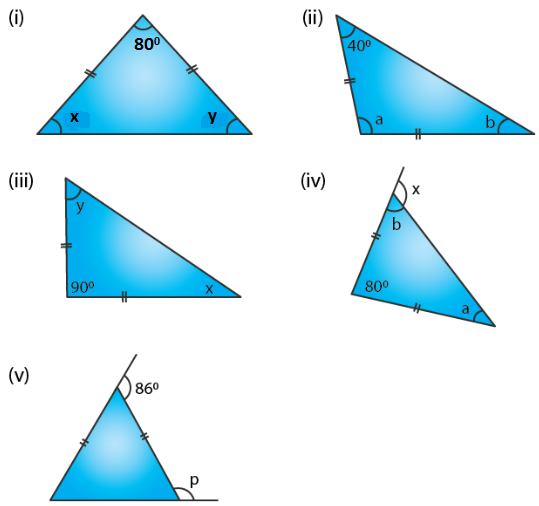Solution:

(i) From the figure (i)

x = y as the angles opposite to equal sides

In a triangle

x + y + 800 = 1800

Substituting the values

x + x + 800 = 1800

By further calculation

2x = 1800 – 800 = 1000

x = 1000/2 = 500

Therefore, x = y = 500.

(ii) From the figure (ii)

b = 400 as the angles opposite to equal sides

In a triangle

a + b + 400 = 1800

Substituting the values

a + 400 + 400 = 1800

By further calculation

a = 180 – 80 = 1000

Therefore, a = 1000 and b = 400.

(iii) From the figure (iii)

x = y as the angles opposite to equal sides

In a triangle

x + y + 900 = 1800

Substituting the values

x + x + 900 = 1800

By further calculation

2x = 180 – 90 = 900

x = 90/2 = 450

Therefore, x = y = 450.

(iv) From the figure (iv)

a = b as the angles opposite to equal sides are equal

In a triangle

a + b + 800 = 1800

Substituting the values

a + a + 800 = 1800

By further calculation

2a = 180 – 80 = 1000

a = 1000/2 = 500

Here a = b = 500

We know that in a triangle the exterior angle is equal to sum of its opposite interior angles

x = a + 800

So we get

x = 50 + 80 = 1300

Therefore, a = 500, b = 500 and x = 1300.

(v) From the figure (v)

In an isosceles triangle consider each equal angle = x

x + x = 860

2x = 860

So we get

x = 860/2 = 430

For a linear pair

p + x = 1800

Substituting the values

p + 430 = 1800

By further calculation

p = 180 – 43 = 1370

Therefore, p = 1370.

2. Apply the properties of isosceles and equilateral triangles to find the unknown angles in the given figures: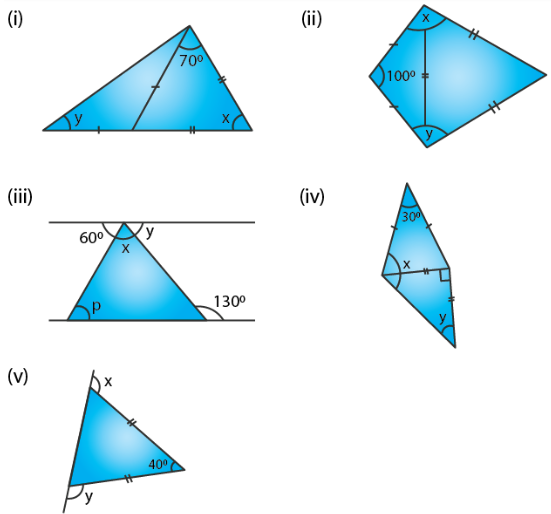Solution:

(i) a = 700 as the angles opposite to equal sides are equal

In a triangle

a + 700 + x = 1800

Substituting the values

700 + 700 + x = 1800

By further calculation

x = 180 – 140 = 400

y = b as the angles opposite to equal sides are equal

Here a = y + b as the exterior angle is equal to sum of interior opposite angles

700 = y + y

So we get

2y = 700

y = 700/2 = 350

Therefore, x = 400 and y = 350.

(ii) From the figure (ii)

Each angle is 600 in an equilateral triangle

In an isosceles triangle

Consider each base angle = a

a + a + 1000 = 1800

By further calculation

2a = 180 – 100 = 800

So we get

a = 800/2 = 400

x = 600 + 400 = 1000

y = 600 + 400 = 1000

(iii) From the figure (iii)

1300 = x + p as the exterior angle is equal to the sum of interior opposite angles

It is given that the lines are parallel

Here p = 600 is the alternate angles and y = a

In a linear pair

a + 1300 = 1800

By further calculation

a = 180 – 130 = 500

Here x + p = 1300

Substituting the values

x + 600 = 1300

By further calculation

x = 130 – 60 = 700

Therefore, x = 700, y = 500 and p = 600.

(iv) From the figure (iv)

x = a + b

Here b = y and a = c as the angles opposite to equal sides are equal

a + c + 300 = 1800

Substituting the values

a + a + 300 = 1800

By further calculation

2a = 180 – 30 = 1500

a = 150/2 = 750

We know that

b + y = 900

Substituting the values

y + y = 900

2y = 900

y = 90/2 = 450

where b = 450

Therefore, x = a + b = 75 + 45 = 1200 and y = 450.

(v) From the figure (v)

a + b + 400 = 1800

So we get

a + b = 180 – 40 = 1400

The angles opposite to equal sides are equal

a = b = 140/2 = 700

x = b + 400 = 700 + 400= 1100

Here the exterior angle of a triangle is equal to the sum of its interior opposite angles

In the same way

y = a + 400

Substituting the values

y = 700 + 400 = 1100

Therefore, x = y = 1100.

3. The angle of vertex of an isosceles triangle is 100°. Find its base angles.

Solution:

Consider ∆ ABC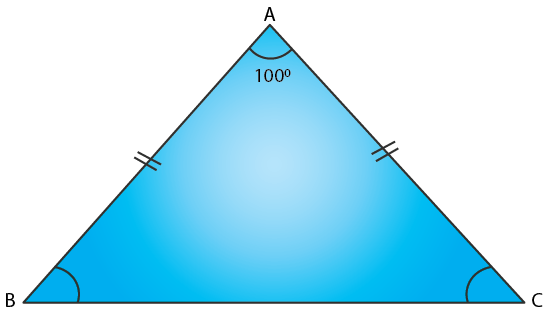Here AB = AC and ∠B = ∠C

We know that

∠A = 1000

In a triangle

∠A + ∠B + ∠C = 1800

Substituting the values

1000 + ∠B + ∠B = 1800

By further calculation

2∠B = 1800 – 1000 = 800

∠B = 80/2 = 400

Therefore, ∠B = ∠C = 400.

4. One of the base angles of an isosceles triangle is 52°. Find its angle of vertex.

Solution:

It is given that the base angles of isosceles triangle ABC = 520

Here ∠B = ∠C = 520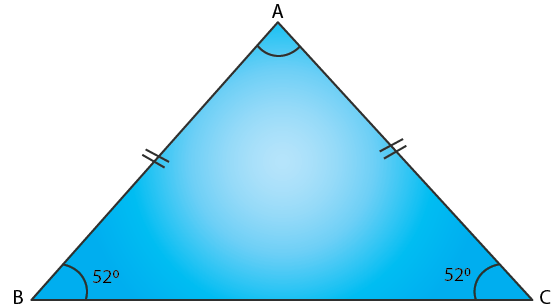In a triangle

∠A + ∠B + ∠C = 1800

Substituting the values

∠A + 520 + 520 = 1800

By further calculation

∠A = 180 – 104 = 760

Therefore, ∠A = 760.

5. In an isosceles triangle, each base angle is four times of its vertical angle. Find all the angles of the triangle.

Solution:

Consider the vertical angle of an isosceles triangle = x

So the base angle = 4x

In a triangle

x + 4x + 4x = 1800

By further calculation

9x = 1800

x = 180/9 = 200

So the vertical angle = 200

Each base angle = 4x = 4 × 200 = 800

6. The vertical angle of an isosceles triangle is 15° more than each of its base angles. Find each angle of the triangle.

Solution:

Consider the angle of the base of isosceles triangle = x0

So the vertical angle = x + 150

In a triangle

x + x + x + 150 = 1800

By further calculation

3x = 180 – 15 = 1650

x = 165/3 = 550

Therefore, the base angle = 550

Vertical angle = 55 + 15 = 700.

7. The base angle of an isosceles triangle is 15° more than its vertical angle. Find its each angle.

Solution:

Consider the vertical angle of the isosceles triangle = x0

Here each base angle = x + 150

In a triangle

x + 150 + x + 150 + x = 1800

By further calculation

3x + 300 = 1800

3x = 180 – 30 = 1500

x = 150/3 = 500

Therefore, vertical angle = 500 and each base angle = 50 + 15 = 650.

8. The vertical angle of an isosceles triangle is three times the sum of its base angles. Find each angle.

Solution:

Consider each base angle of an isosceles triangle = x

Vertical angle = 3 (x + x) = 3 (2x) = 6x

In a triangle

6x + x + x = 1800

By further calculation

8x = 1800

x = 180/8 = 22.50

Therefore, each base angle = 22.50 and vertical angle = 3 (22.5 + 22.5) = 3 × 45 = 1350.

9. The ratio between a base angle and the vertical angle of an isosceles triangle is 1 : 4. Find each angle of the triangle.

Solution:

It is given that the ratio between a base angle and the vertical angle of an isosceles triangle = 1: 4

Consider base angle = x

Vertical angle = 4x

In a triangle

x + x + 4x = 1800

By further calculation

6x = 1800

x = 180/6 = 300

Therefore, each base angle = x = 300 and vertical angle = 4x = 4 × 300 = 1200.

10. In the given figure, BI is the bisector of ∠ABC and CI is the bisector of ∠ACB. Find ∠BIC.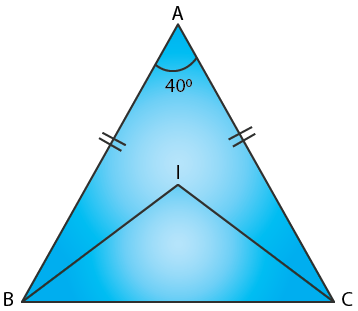Solution:

In ∆ ABC

BI is the bisector of ∠ABC and CI is the bisector of ∠ACB

Here AB = AC

∠B = ∠C as the angles opposite to equal sides are equal

We know that ∠A = 400In a triangle

∠A + ∠B + ∠C = 1800

Substituting the values

400 + ∠B + ∠B = 1800

By further calculation

400 + 2∠B = 1800

2∠B = 180 – 40 = 1400

∠B = 140/2 = 700

Here BI and CI are the bisectors of ∠ABC and ∠ACB

∠IBC = ½ ∠ABC = ½ × 700 = 350

∠ICB = ½ ∠ACB = ½ × 700 = 350

In ∆ IBC

∠BIC + ∠IBC + ∠ICB = 1800

Substituting the values

∠BIC + 350 + 350 = 1800

By further calculation

∠BIC = 180 – 70 = 1100

Therefore, ∠BIC = 1100.

11. In the given figure, express a in terms of b.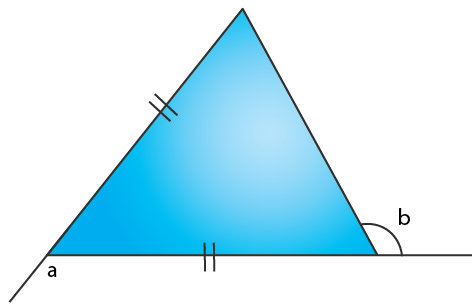Solution:

From the ∆ ABC

BC = BA

∠BCA = ∠BAC

Here the exterior ∠CBE = ∠BCA + ∠BAC

a = ∠BCA + ∠BCA

a = 2∠BCA …… (1)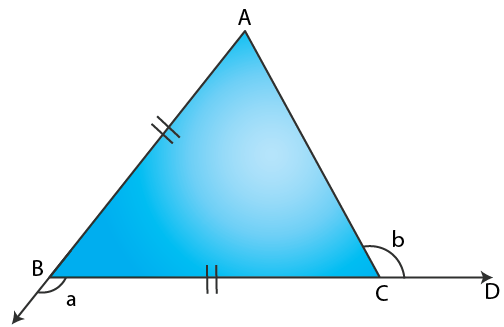Here ∠ACB = 1800 – b

Where ∠ACD and ∠ACB are linear pair

∠BCA = 1800 – b ……. (2)

We get

a = 2 ∠BCA

Substituting the values

a = 2 (1800 – b)

a = 3600 – 2b

12. (a) In Figure (i) BP bisects ∠ABC and AB = AC. Find x.
(b) Find x in Figure (ii) Given: DA = DB = DC, BD bisects ∠ABC and ∠ADB = 70°.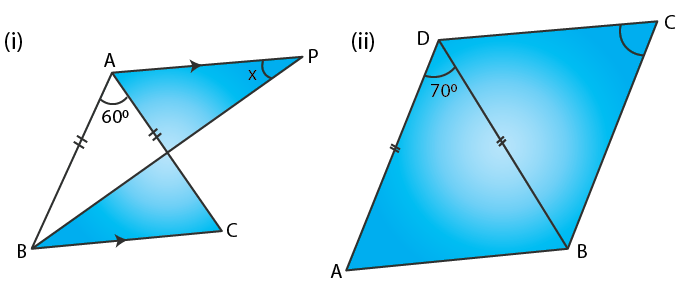Solution:

(a) From the figure (i)

AB = AC and BP bisects ∠ABC

AP is drawn parallel to BC

Here PB is the bisector of ∠ABC

∠PBC = ∠PBA

∠APB = ∠PBC are alternate angles

x = ∠PBC ….. (1)

In ∆ ABC

∠A = 600

Since AB = AC we get ∠B = ∠C

In a triangle

∠A + ∠B + ∠C = 1800

Substituting the values

600 + ∠B + ∠C = 1800

We get

600 + ∠B + ∠B = 1800

By further calculation

2∠B = 180 – 60 = 1200

∠B = 120/2 = 600

½ ∠B = 60/2 = 300

∠PBC = 300

So from figure (i) x = 300

(b) From the figure (ii)

DA = DB = DC

Here BD bisects ∠ABC and ∠ADB = 700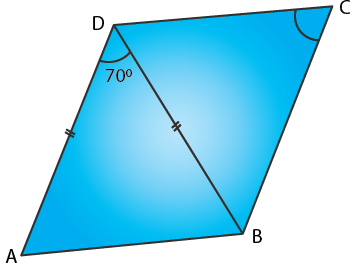In a triangle

∠ADB + ∠DAB + ∠DBA = 1800

Substituting the values

700 + ∠DBA + ∠DBA = 1800

By further calculation

700 + 2∠DBA = 1800

2∠DBA = 180 – 70 = 1100

∠DBA = 110/2 = 550

Here BD is the bisector of ∠ABC

So ∠DBA = ∠DBC = 550

In ∆ DBC

DB = DC

∠DCB = ∠DBC

Hence, x = 550.

13. In each figure, given below, ABCD is a square and ∆ BEC is an equilateral triangle.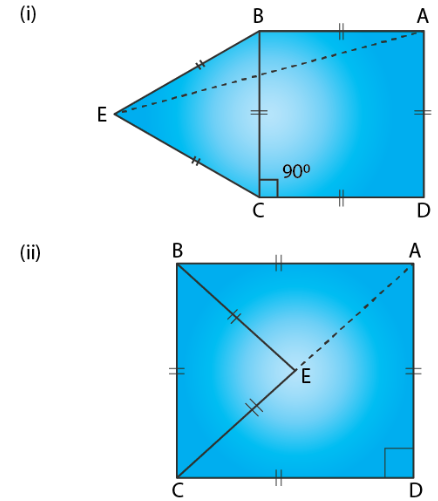Find, in each case: (i) ∠ABE (ii) ∠BAE

Solution:

The sides of a square are equal and each angle is 900

In an equilateral triangle all three sides are equal and all angles are 600

In figure (i) ABCD is a square and ∆ BEC is an equilateral triangle

(i) ∠ABE = ∠ABC + ∠CBE

Substituting the values

∠ABE = 900 + 600= 1500

(ii) In ∆ ABE

∠ABE + ∠BEA + ∠BAE = 1800

Substituting the values

1500 + ∠BAE + ∠BAE = 1800

By further calculation

2∠BAE = 180 – 150 = 300

∠BAE = 30/2 = 150

In figure (ii) ABCD is a square and ∆ BEC is an equilateral triangle

(i) ∠ABE = ∠ABC – ∠CBE

Substituting the values

∠ABE = 900 – 600 = 300

(ii) In ∆ ABE

∠ABE + ∠BEA + ∠BAE = 1800

Substituting the values

300 + ∠BAE + ∠BAE = 1800

By further calculation

2∠BAE = 180 – 30 = 1500

∠BAE = 150/2 = 750

14. In ∆ ABC, BA and BC are produced. Find the angles a and h. if AB = BC.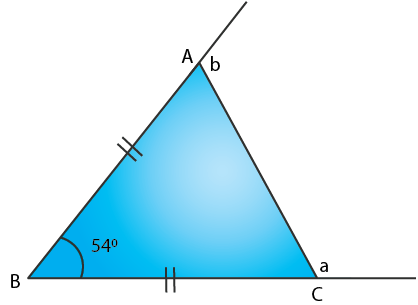Solution:

In ∆ ABC, BA and BC are produced

∠ABC = 540 and AB = BC

In ∆ ABC

∠BAC + ∠BCA + ∠ABC = 1800

Substituting the values

∠BAC + ∠BAC + 540 = 1800

2∠BAC = 180 – 54 = 1260

∠BAC = 126/2 = 630

∠BCA = 630

In a linear pair

∠BAC + b = 1800

Substituting the value

630 + b = 1800

So we get

b = 180 – 63 = 1170

In a linear pair

∠BCA + a = 1800

Substituting the value

630 + a = 1800

So we get

a = 180 – 63 = 1170

Therefore, a = b = 1170.

#### Exercise 15C page: 185

1. Construct a ∆ABC such that:
(i) AB = 6 cm, BC = 4 cm and CA = 5.5 cm
(ii) CB = 6.5 cm, CA = 4.2 cm and BA = 51 cm
(iii) BC = 4 cm, AC = 5 cm and AB = 3.5 cm

Solution:

(i) Steps of Construction

1. Construct a line segment BC = 4 cm.

2. Taking B as centre and 6 cm as radius construct an arc.

3. Taking C as centre and 5.5 cm as radius construct another arc which intersects the first arc at the point A.

4. Now join AB and AC.

Therefore, ∆ABC is the required triangle.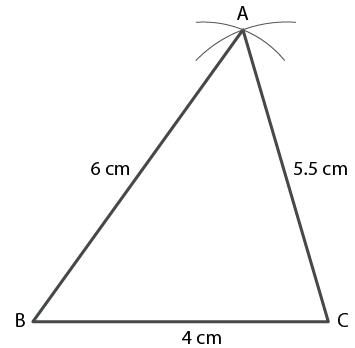(ii) Steps of Construction

1. Construct a line segment CB = 6.5 cm

2. Taking C as centre and 4.2 cm as radius construct an arc.

3. Taking B as centre and 5.1 cm as radius construct another arc which intersects the first arc at the point A.

4. Now join AC and AB.

Therefore, ∆ABC is the required triangle.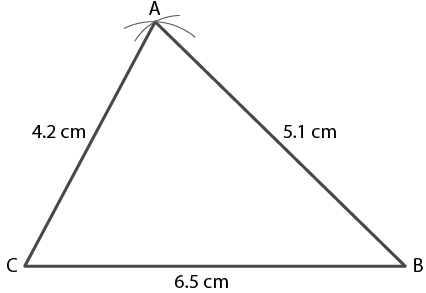(iii) Steps of Construction

1. Construct a line segment BC = 4 cm

2. Taking B as centre and 3.5 cm as radius construct an arc.

3. Taking C as centre and 5 cm as radius construct another arc which intersects the first arc at the point A.

4. Now join AB and AC.

Therefore, ∆ABC is the required triangle.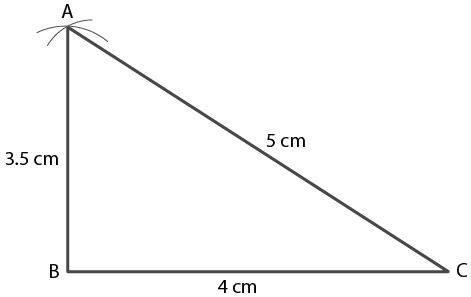2. Construct a ABC such that:
(i) AB = 7 cm, BC = 5 cm and ∠ABC = 60°
(ii) BC = 6 cm, AC = 5.7 cm and ∠ACB = 75°
(iii) AB = 6.5 cm, AC = 5.8 cm and ∠A = 45°

Solution:

(i) Steps of Construction

1. Construct a line segment AB = 7 cm.

2. At the point B construct a ray which makes an angle 600 and cut off BC = 5cm.

3. Now join AC.

Therefore, ∆ABC is the required triangle.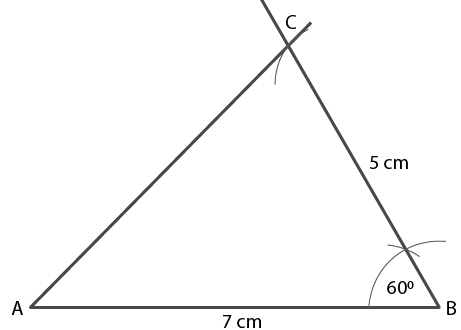(ii) Steps of Construction

1. Construct a line segment BC = 6 cm.

2. At the point C construct a ray which makes an angle 750 and cut off CA = 5.7 cm.

3. Now join AB.

Therefore, ∆ABC is the required triangle.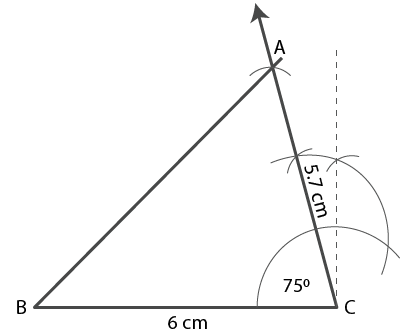(iii) Steps of Construction

1. Construct a line segment AB = 6.5 cm.

2. At the point A construct a ray which makes an angle 450 and cut off AC = 5.8 cm.

3. Now join CB.

Therefore, ∆ABC is the required triangle.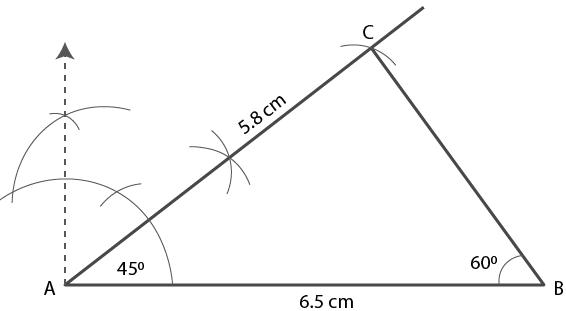3. Construct a ∆ PQR such that :
(i) PQ = 6 cm, ∠Q = 60° and ∠P = 45°. Measure ∠R.
(ii) QR = 4.4 cm, ∠R = 30° and ∠Q = 75°. Measure PQ and PR.
(iii) PR = 5.8 cm, ∠P = 60° and ∠R = 45°.
Measure ∠Q and verify it by calculations

Solution:

(i) Steps of Construction

1. Construct a line segment PQ = 6 cm.

2. At point P construct a ray which makes an angle 450.

3. At point Q construct another ray which makes an angle 600 which intersect the first ray at point R.

Therefore, ∆ PQR is the required triangle.

By measuring ∠R = 750.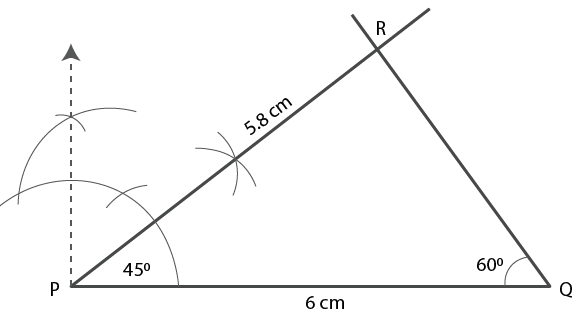(ii) Steps of Construction

1. Construct a line segment QR = 4.4 cm.

2. At point Q construct a ray which makes an angle 750.

3. At point R construct another ray which makes an angle 300 which intersect the first ray at point R.

Therefore, ∆ PQR is the required triangle.

By measuring the length, PQ = 2.1 cm and PR = 4.4 cm.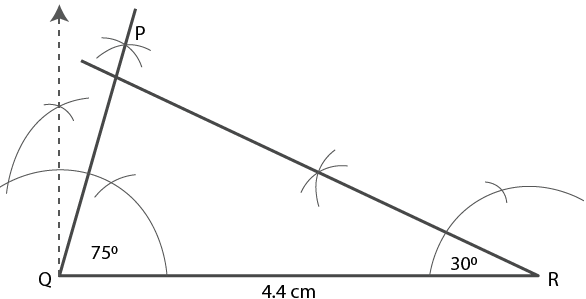(iii) Steps of Construction

1. Construct a line segment PR = 5.8 cm.

2. At point P construct a ray which makes an angle 600.

3. At point R construct another ray which makes an angle 450 which intersect the first ray at point Q.

Therefore, ∆ PQR is the required triangle.

By measuring ∠Q = 750.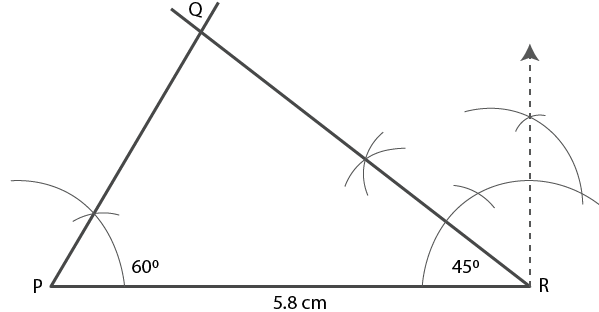Verification –

∠P + ∠Q + ∠R = 1800

Substituting the values

600 + ∠Q + 450 = 1800

By further calculation

∠Q = 180 – 105 = 750

4. Construct an isosceles ABC such that:
(i) base BC = 4 cm and base angle = 30°
(ii) base AB = 6.2 cm and base angle = 45°
(iii) base AC = 5 cm and base angle = 75°.
Measure the other two sides of the triangle.

Solution:

(i) Steps of Construction

In an isosceles triangle the base angles are equal

1. Construct a line segment BC = 4 cm.

2. At the points B and C construct rays which makes an angle 300 intersecting each other at the point A.

Therefore, ∆ ABC is the required triangle.

By measuring the equal sides, each is 2.5 cm in length approximately.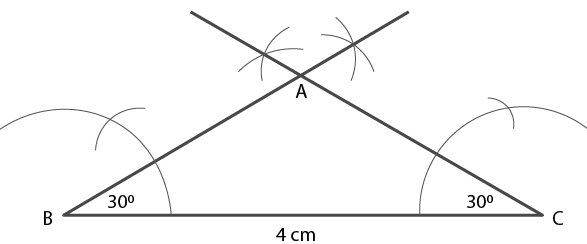(ii) Steps of Construction

In an isosceles triangle the base angles are equal

1. Construct a line segment AB = 6.2 cm.

2. At the points A and B construct rays which makes an angle 450 intersecting each other at the point C.

Therefore, ∆ ABC is the required triangle.

By measuring the equal sides, each is 4.3 cm in length approximately.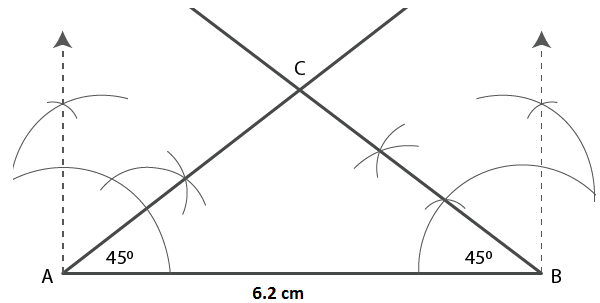(iii) Steps of Construction

In an isosceles triangle the base angles are equal

1. Construct a line segment AC = 5 cm.

2. At the points A and C construct rays which makes an angle 750 intersecting each other at the point B.

Therefore, ∆ ABC is the required triangle.

By measuring the equal sides, each is 9.3 cm in length approximately.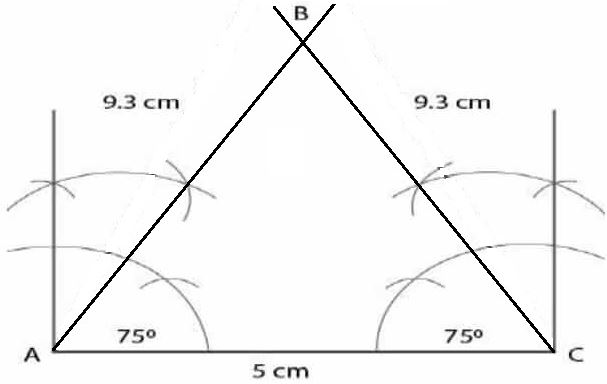5. Construct an isosceles ∆ABC such that:
(i) AB = AC = 6.5 cm and ∠A = 60°
(ii) One of the equal sides = 6 cm and vertex angle = 45°. Measure the base angles.
(iii) BC = AB = 5-8 cm and ZB = 30°. Measure ∠A and ∠C.

Solution:

(i) Steps of Construction

1. Construct a line segment AB = 6.5 cm.

2. At point A construct a ray which makes an angle 600.

3. Now cut off AC = 6.5cm

4. Join BC.

Therefore, ∆ ABC is the required triangle.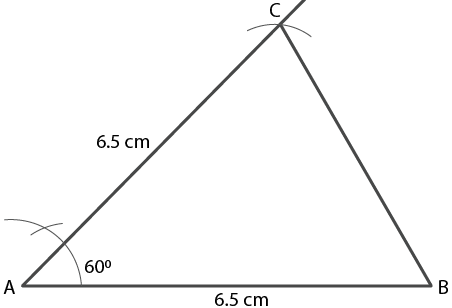(ii) Steps of Construction

1. Construct a line segment AB = 6 cm.

2. At point A construct a ray which makes an angle 450.

3. Now cut off AC = 6cm

4. Join BC.

Therefore, ∆ ABC is the required triangle.

By measuring ∠B and ∠C, both are equal to 67 ½ 0.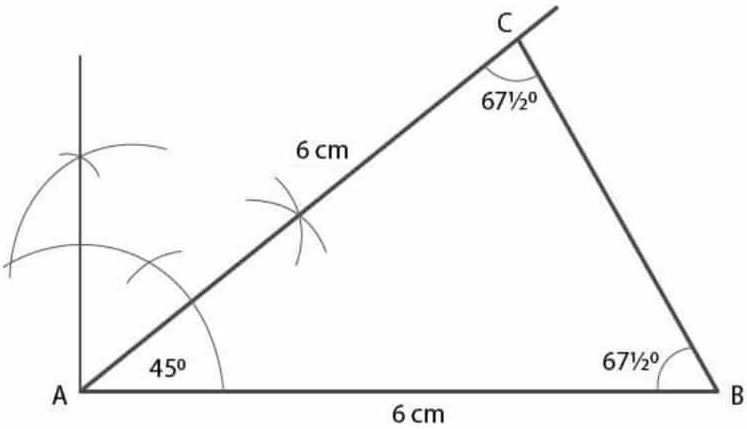(iii) Steps of Construction

1. Construct a line segment BC = 5.8 cm.

2. At point B construct a ray which makes an angle 300.

3. Now cut off BA = 5.8cm

4. Join AC.

Therefore, ∆ ABC is the required triangle.

By measuring ∠C and ∠A is equal to 750.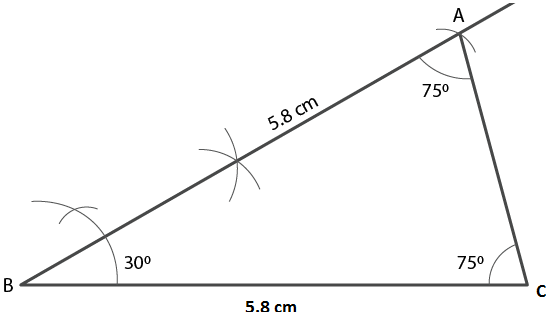6. Construct an equilateral triangle ABC such that:
(i) AB = 5 cm. Draw the perpendicular bisectors of BC and AC. Let P be the point of intersection of these two bisectors. Measure PA, PB and PC.
(ii) Each side is 6 cm.

Solution:

(i) Steps of Construction

1. Construct a line segment AB = 5cm.

2. Taking A and B as centres and 5 cm radius, construct two arcs which intersect each other at the point C.

3. Now join AC and BC where ∆ ABC is the required triangle.

4. Construct perpendicular bisectors of sides AC and BC which intersect each other at the point p.

5. Join PA, PB and PC.

By measuring each is 2.8 cm.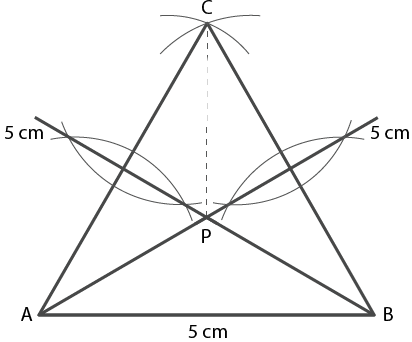(ii) Steps of Construction

1. Construct a line segment AB = 6cm.

2. Taking A and B as centres and 6 cm radius, construct two arcs which intersect each other at the point C.

3. Now join AC and BC

Therefore, ∆ ABC is the required triangle.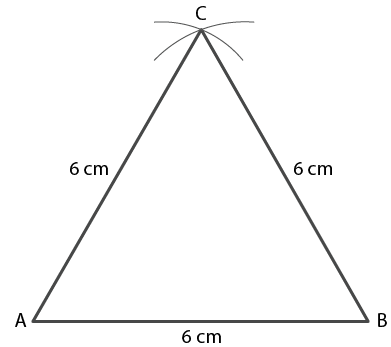7. (i) Construct a ∆ ABC such that AB = 6 cm, BC = 4.5 cm and AC = 5.5 cm. Construct a circumcircle of this triangle.
(ii) Construct an isosceles ∆PQR such that PQ = PR = 6.5 cm and ∠PQR = 75°. Using ruler and compasses only construct a circumcircle to this triangle.
(iii) Construct an equilateral triangle ABC such that its one side = 5.5 cm.
Construct a circumcircle to this triangle.

Solution:

(i) Steps of Construction

1. Construct a line segment BC = 4.5 cm.

2. Taking B as centre and 6 cm radius construct an arc.

3. Taking C as centre and 5.5 cm radius construct another arc which intersects the first arc at point A.

4. Now join AB and AC

Therefore, ∆ ABC is the required triangle.

5. Construct a perpendicular bisector of AB and AC which intersect each other at the point O.

6. Now join OB, OC and OA.

7. Taking O as centre and radius OA construct a cirlce which passes through the points A, B and C.

This is the required circumcircle of ∆ ABC.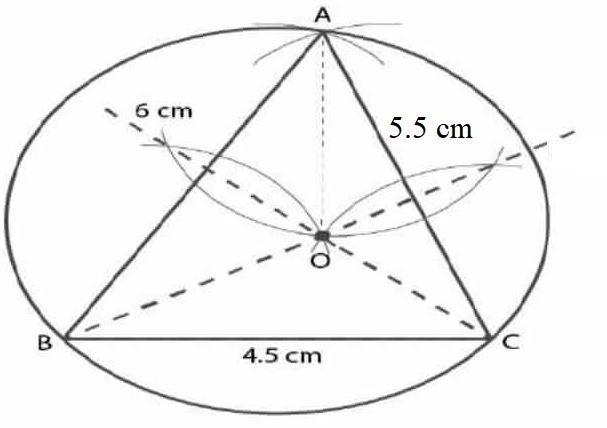(ii) Steps of Construction

1. Construct a line segment PQ = 6.5 cm.

2. At point Q, construct an arc which makes an angle 750.

3. Taking P as centre and radius 6.5 cm construct an arc which intersects the angle at point R.

4. Join PR.

∆ PQR is the required triangle.

4. Construct the perpendicular bisector of sides PQ and PR which intersects each other at the point O.

5. Join OP, OQ and OR.

6. Taking O as centre and radius equal to OP or OQ or OR construct a circle which passes through P, Q and R.

This is the required circumcircle of ∆ PQR.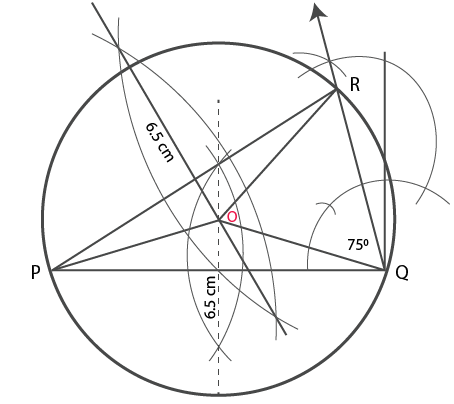(iii) Steps of Construction

1. Construct a line segment AB = 5.5 cm.

2. Taking A and B as centres and radius 5.5 cm construct two arcs which intersect each other at point C.

3. Now join AC and BC.

∆ ABC is the required triangle.

4. Construct perpendicular bisectors of sides AC and BC which intersect each other at the point O.

5. Now join OA, OB and OC.

6. Taking O as centre and OA or OB or OC as radius construct a circle which passes through A, B and C.

This is the required circumcircle.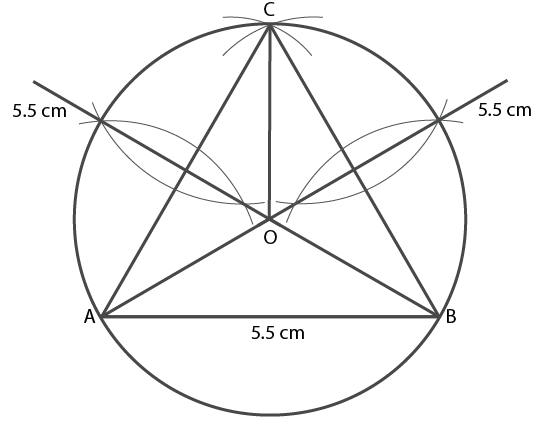8. (i) Construct a ∆ABC such that AB = 6 cm, BC = 5.6 cm and CA = 6.5 cm. Inscribe a circle to this triangle and measure its radius.
(ii) Construct an isosceles ∆ MNP such that base MN = 5.8 cm, base angle MNP = 30°. Construct an incircle to this triangle and measure its radius.
(iii) Construct an equilateral ∆DEF whose one side is 5.5 cm. Construct an incircle to this triangle.
(iv) Construct a ∆ PQR such that PQ = 6 cm, ∠QPR = 45° and angle PQR = 60°. Locate its incentre and then draw its incircle.

Solution:

(i) Steps of Construction

1. Construct a line segment AB = 6 cm.

2. Taking A as centre and 6.5 cm as radius and B as centre and 5.6 cm as radius construct arcs which intersect each other at point C.

3. Now join AC and BC.

4. Construct the angle bisector of ∠A and ∠B which intersect each other at point I.

5. From the point I construct IL which is perpendicular to AB.

6. Taking I as centre and IL as radius construct a circle which touches the sides of ∆ABC internally.

By measuring the required incircle the radius is 1.6 cm.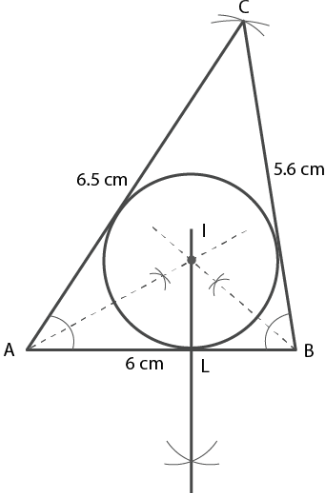(ii) Steps of Construction

1. Construct a line segment MN = 5.8 cm.

2. At points M and N construct two rays which make an angle 300 each intersecting each other at point P.

3. Construct the angle bisectors of ∠M and ∠N which intersect each other at point I.

4. From the point I draw perpendicular IL on MN.

5. Taking I as centre and IL as radius construct a circle which touches the sides of ∆ PMB internally.

By measuring the required incircle the radius is 0.6 cm.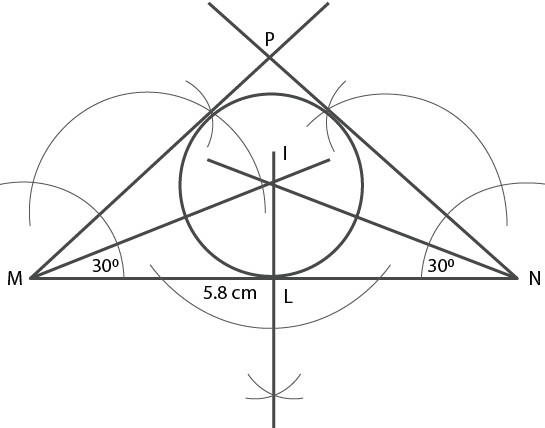(iii) Steps of Construction

1. Construct a line segment BC = 5.5 cm.

2. Taking B and C as centres and 5.5 cm radius construct two arcs which intersect each other at point A.

3. Now join AB and AC.

4. Construct the perpendicular bisectors of ∠B and ∠C which intersect each other at the point I.

5. From the point I construct IL which is perpendicular to BC.

6. Taking I as centre and IL as radius construct a circle which touches the sides of ∆ABC internally.

This is the required incircle.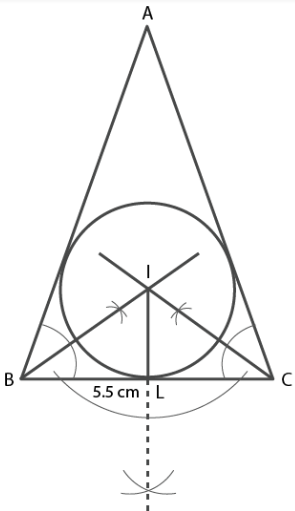(iv) Steps of Construction

1. Construct a line segment PQ = 6 cm.

2. At the point P construct rays which make an angle of 450 and at point Q which makes an angle 600 thats intersects each other at point R.

3. Construct the bisectors of ∠P and ∠Q which intersect each other at point I.

4. From the point I construct IL which is perpendicular to PQ.

5. Taking I as centre and IL as radius construct a circle which touches the sides of ∆PQR internally.

This is the required incircle where the point I is incentre.